### Reverse-engineering the Globus INK, a Soviet spaceflight navigation computer

One of the most interesting navigation instruments onboard Soyuz spacecraft was the Globus INK,1 which used a rotating globe to indicate the spacecraft's position above the Earth. This electromechanical analog computer used an elaborate system of gears, cams, and differentials to compute the spacecraft's position. The globe rotates in two dimensions: it spins end-over-end to indicate the spacecraft's orbit, while the globe's hemispheres rotate according to the Earth's daily rotation around its axis.2 The spacecraft's position above the Earth was represented by the fixed crosshairs on the plastic dome. The Globus also has latitude and longitude dials next to the globe to show the position numerically, while the light/shadow dial below the globe indicated when the spacecraft would enter or leave the Earth's shadow.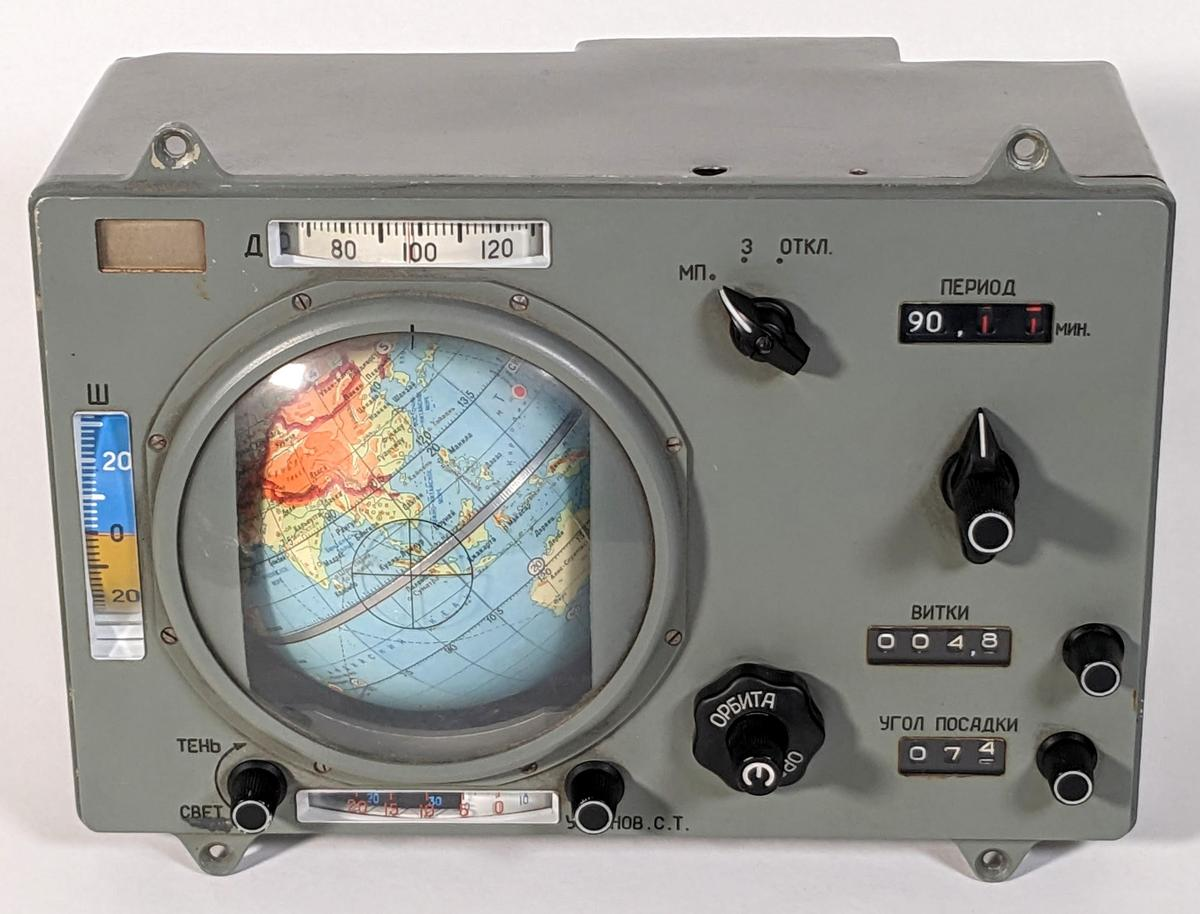The INK-2S "Globus" space navigation indicator.

Opening up the Globus reveals that it is packed with complicated gears and mechanisms. It's amazing that this mechanical technology was used from the 1960s into the 21st century. But what are all those gears doing? How can orbital functions be implemented with gears? To answer these questions, I reverse-engineered the Globus and traced out its system of gears.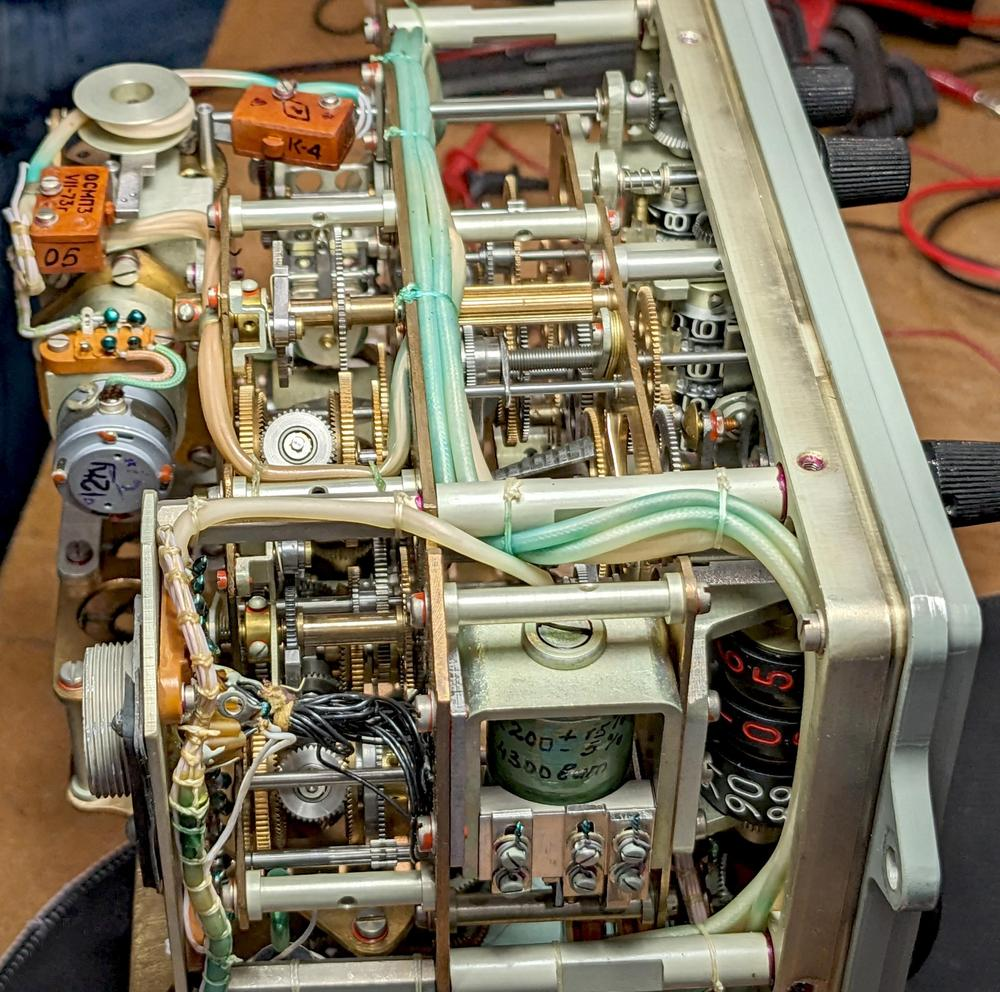The Globus with the case removed, showing the complex gearing inside.

The diagram below summarizes my analysis. The Globus is an analog computer that represents values by rotating shafts by particular amounts. These rotations control the globe and the indicator dials. The flow of these rotational signals is shown by the lines on the diagram. The computation is based around addition, performed by ten differential gear assemblies. On the diagram, each "⨁" symbol indicates one of these differential gear assemblies. Other gears connect the components while scaling the signals through various gear ratios. Complicated functions are implemented with three specially-shaped cams. In the remainder of this blog post, I will break this diagram down into functional blocks and explain how the Globus operates.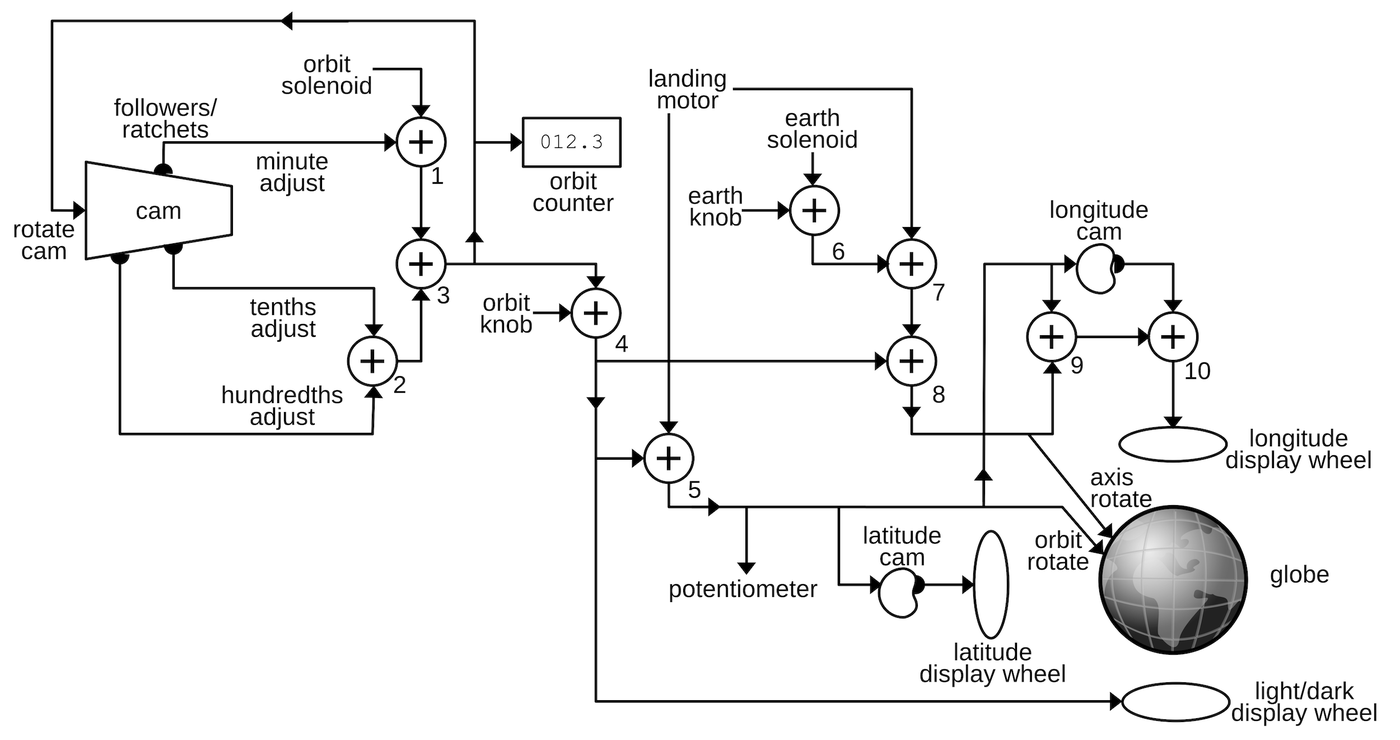This diagram shows the interconnections of the gear network in the Globus.

For all its complexity, though, the functionality of the Globus is pretty limited. It only handles a fixed orbit at a specific angle, and treats the orbit as circular. The Globus does not have any navigation input such as an inertial measurement unit (IMU). Instead, the cosmonauts configured the Globus by turning knobs to set the spacecraft's initial position and orbital period. From there, the Globus simply projected the current position of the spacecraft forward, essentially dead reckoning.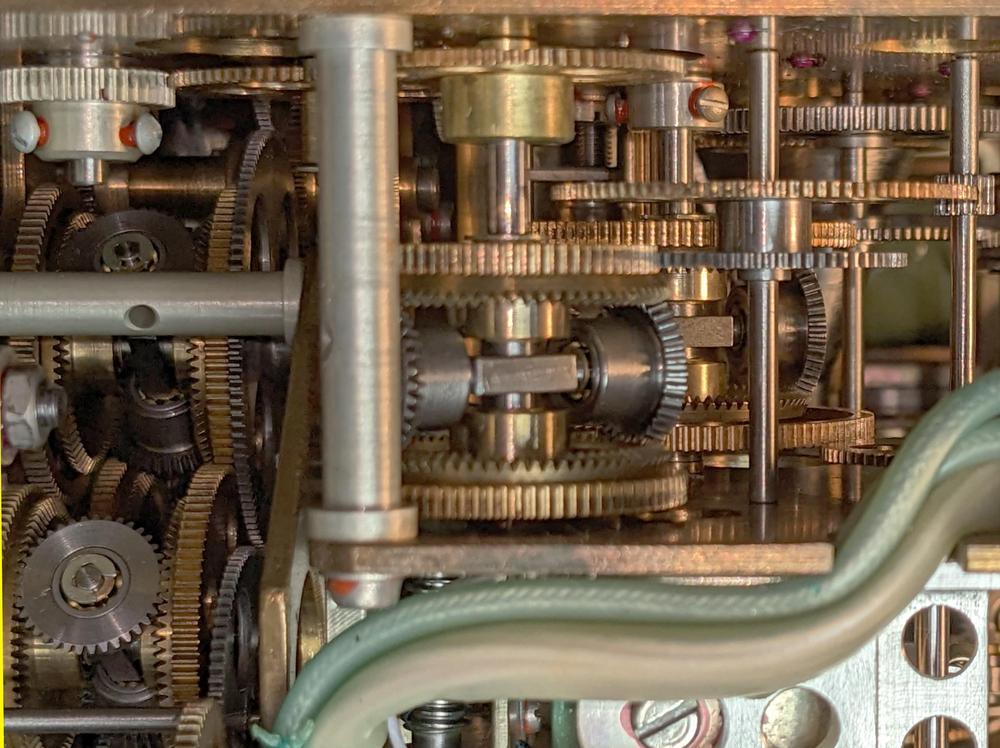A closeup of the gears inside the Globus.

## The globe

On seeing the Globus, one might wonder how the globe is rotated. It may seem that the globe must be free-floating so it can rotate in two axes. Instead, a clever mechanism attaches the globe to the unit. The key is that the globe's equator is a solid piece of metal that rotates around the horizontal axis of the unit. A second gear mechanism inside the globe rotates the globe around the North-South axis. The two rotations are controlled by concentric shafts that are fixed to the unit. Thus, the globe has two rotational degrees of freedom, even though it is attached at both ends.

The photo below shows the frame that holds and controls the globe. The dotted axis is fixed horizontally in the unit and rotations are fed through the two gears at the left. One gear rotates the globe and frame around the dotted axis, while the gear train causes the globe to rotate around the vertical polar axis (while the equator remains fixed).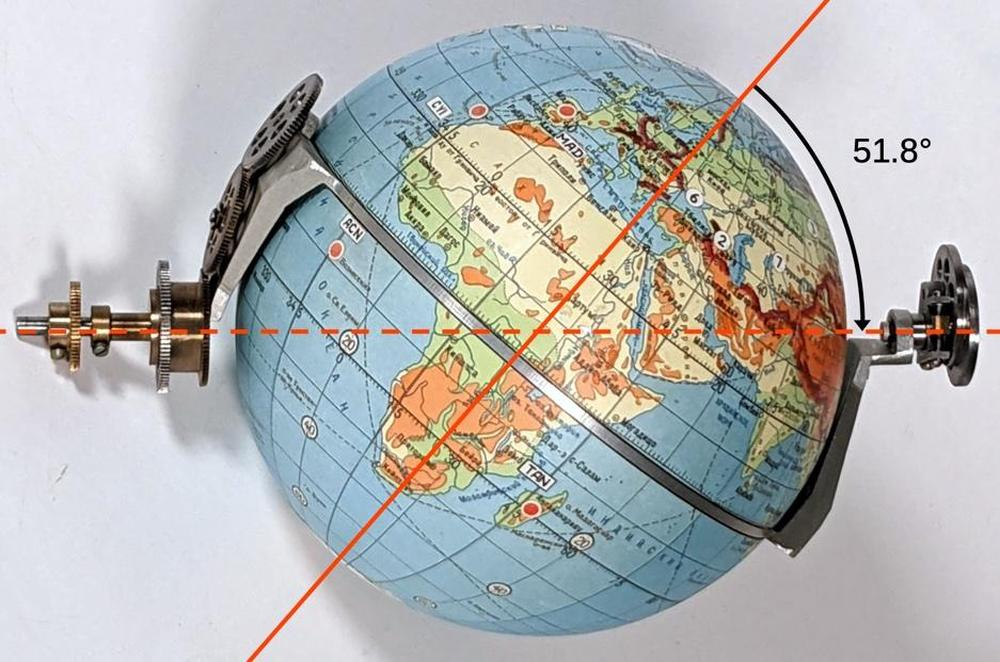The axis of the globe is at 51.8° to support that orbital inclination.

The angle above is 51.8° which is very important: this is the inclination of the standard Soyuz orbit. As a result, simply rotating the globe around the dotted line causes the crosshair to trace the orbit.3 Rotating the two halves of the globe around the poles yields the different paths over the Earth's surface as the Earth rotates. An important consequence of this design is that the Globus only supports a circular orbit at a fixed angle.

## Differential gear mechanism

The primary mathematical element of the Globus is the differential gear mechanism, which can perform addition or subtraction. A differential gear takes two rotations as inputs and produces the (scaled) sum of the rotations as the output. The photo below shows one of the differential mechanisms. In the middle, the spider gear assembly (red box) consists of two bevel gears that can spin freely on a vertical shaft. The spider gear assembly as a whole is attached to a horizontal shaft, called the spider shaft. At the right, the spider shaft is attached to a spur gear (a gear with straight-cut teeth). The spider gear assembly, the spider shaft, and the spider's spur gear rotate together as a unit.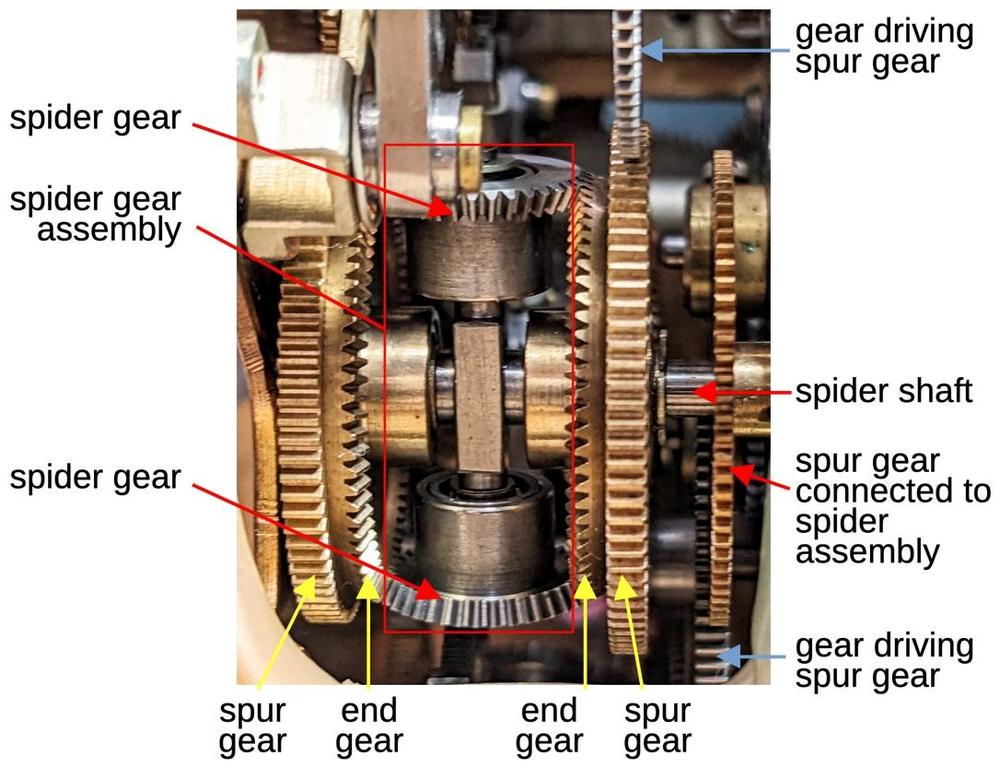Diagram showing the components of a differential gear mechanism.

At the left and right are two end gear assemblies (yellow). The end gear is a bevel gear with angled teeth to mesh with the spider gears. Each end gear is locked to a spur gear and these gears spin freely on the horizontal spider shaft. In total, there are three spur gears: two connected to the end gears and one connected to the spider assembly. In the diagrams, I'll use the symbol below to represent the differential gear assembly: the end gears are symmetric on the top and bottom, with the spider shaft on the side. Any of the three spur gears can be used as an output, with the other two serving as inputs.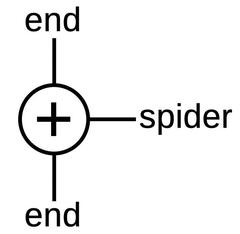The symbol for the differential gear assembly.

To understand the behavior of the differential, suppose the two end gears are driven in the same direction at the same rate, say upwards.4 These gears will push on the spider gears and rotate the spider gear assembly, with the entire differential rotating as a fixed unit. On the other hand, suppose the two end gears are driven in opposite directions. In this case, the spider gears will spin on their shaft, but the spider gear assembly will remain stationary. In either case, the spider gear assembly motion is the average of the two end gear rotations, that is, the sum of the two rotations divided by 2. (I'll ignore the factor of 2 since I'm ignoring all the gear ratios.) If the operation of the differential is still confusing, this vintage Navy video has a detailed explanation.

## The controls and displays

The diagram below shows the controls and displays of the Globus. The rotating globe is the centerpiece of the unit. Its plastic cover has a crosshair that represents the spacecraft's position above the Earth's surface. Surrounding the globe itself are dials that show the longitude, latitude, and the time before entering light and shadow. The cosmonauts manually initialize the globe position with the concentric globe rotation knobs: one rotates the globe along the orbital path while the other rotates the hemispheres. The mode switch at the top selects between the landing position mode, the standard Earth orbit mode, and turning off the unit. The orbit time adjustment configures the orbital time period in minutes while the orbit counter below it counts the number of orbits. Finally, the landing point angle sets the distance to the landing point in degrees of orbit.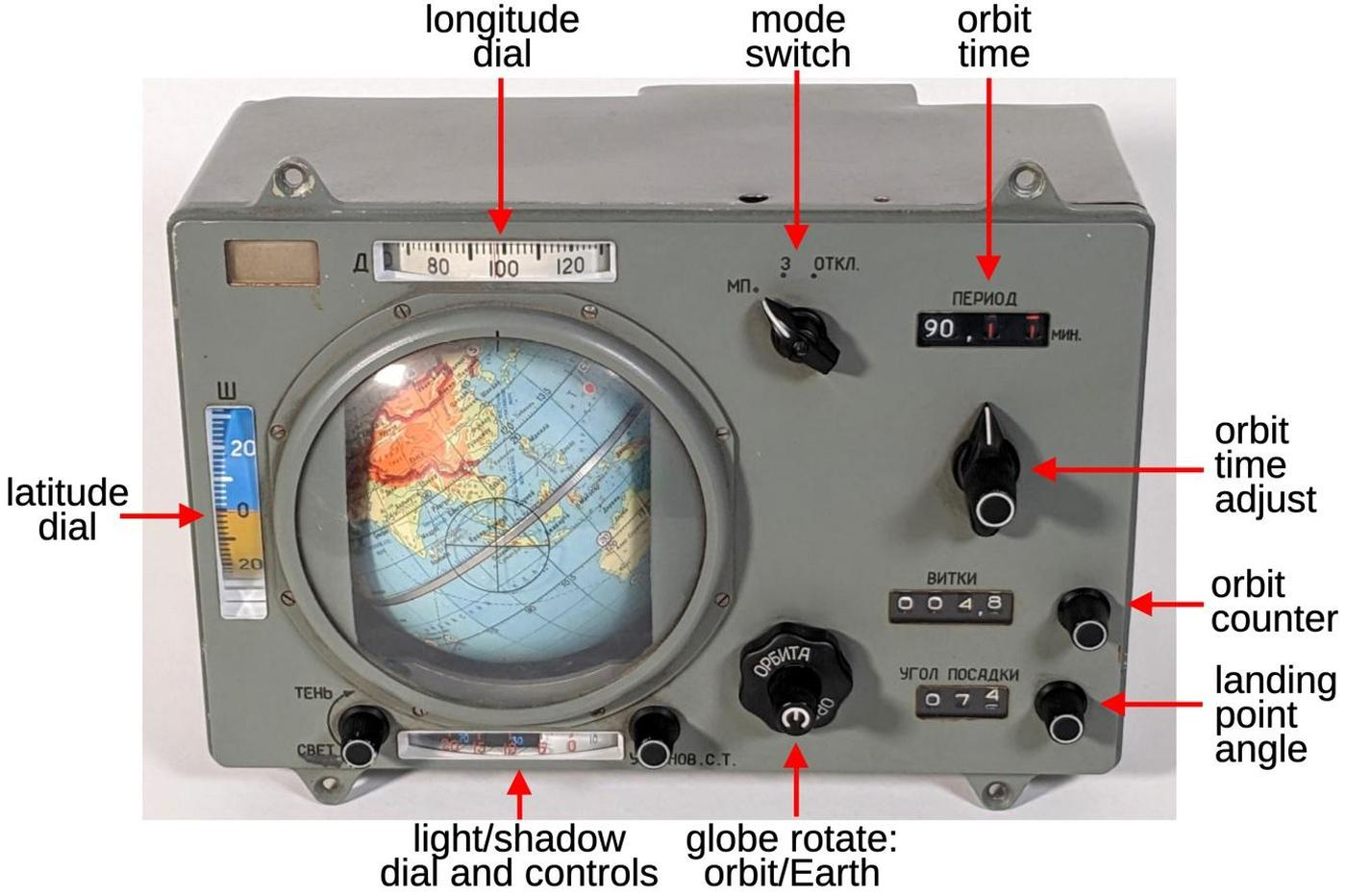The Globus with the controls labeled.

## Computing the orbit time

The primary motion of the Globus is the end-over-end rotation of the globe showing the movement of the spacecraft in orbit. The orbital motion is powered by a solenoid at the top of the Globus that receives pulses once a second and advances a ratchet wheel (video).5 This wheel is connected to a complicated cam and differential system to provide the orbital motion.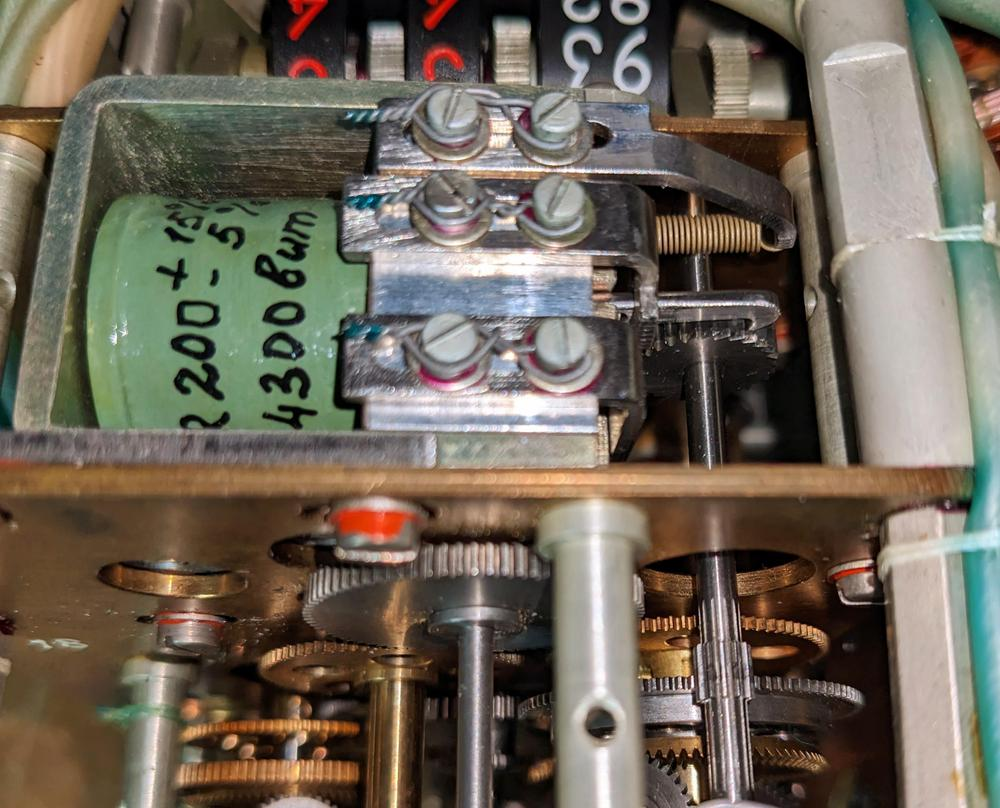The orbit solenoid (green) has a ratchet that rotates the gear to the right. The shaft connects it to differential gear assembly 1 at the bottom right.

Each orbit takes about 92 minutes, but the orbital time can be adjusted by a few minutes in steps of 0.01 minutes6 to account for changes in altitude. The Globus is surprisingly inflexible and this is the only orbital parameter that can be adjusted.7 The orbital period is adjusted by the three-position orbit time switch, which points to the minutes, tenths, or hundredths. Turning the central knob adjusts the indicated period dial.

The problem is how to generate the variable orbital rotation speed from the fixed speed of the solenoid. The solution is a special cam, shaped like a cone with a spiral cross-section. Three followers ride on the cam, so as the cam rotates, the follower is pushed outward and rotates on its shaft. If the follower is near the narrow part of the cam, it moves over a small distance and has a small rotation. But if the follower is near the wide part of the cam, it moves a larger distance and has a larger rotation. Thus, by moving the follower to a particular point on the cam, the rotational speed of the follower is selected. One follower adjusts the speed based on the minutes setting with others for the tenths and hundredths of minutes.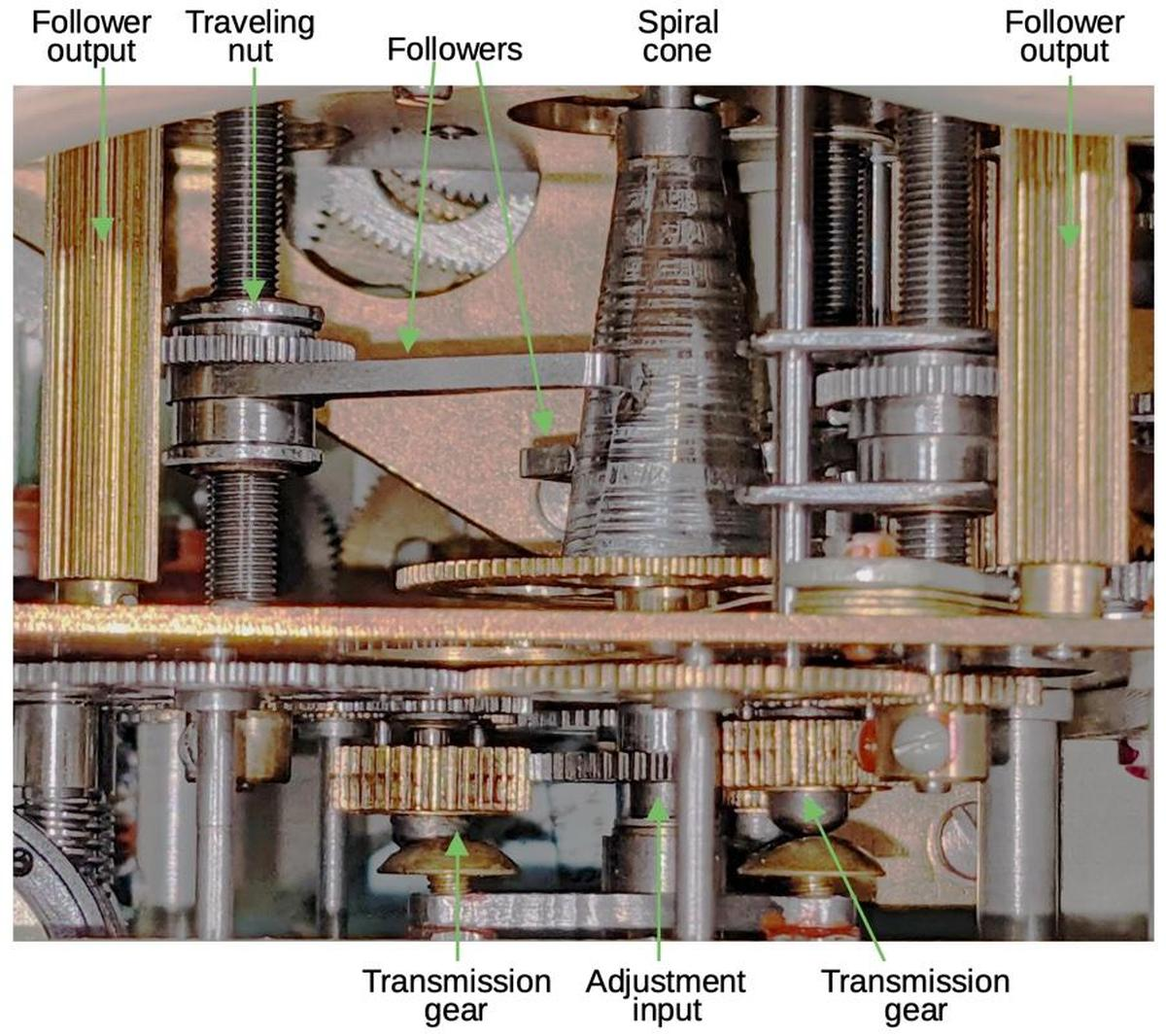A diagram showing the orbital speed control mechanism. The cone has three followers, but only two are visible from this angle. The "transmission" gears are moved in and out by the outer knob to select which follower is adjusted by the inner knob.

Of course, the cam can't spiral out forever. Instead, at the end of one revolution, its cross-section drops back sharply to the starting diameter. This causes the follower to snap back to its original position. To prevent this from jerking the globe backward, the follower is connected to the differential gearing via a slip clutch and ratchet. Thus, when the follower snaps back, the ratchet holds the drive shaft stationary. The drive shaft then continues its rotation as the follower starts cycling out again. Each shaft output is accordingly a (mostly) smooth rotation at a speed that depends on the position of the follower.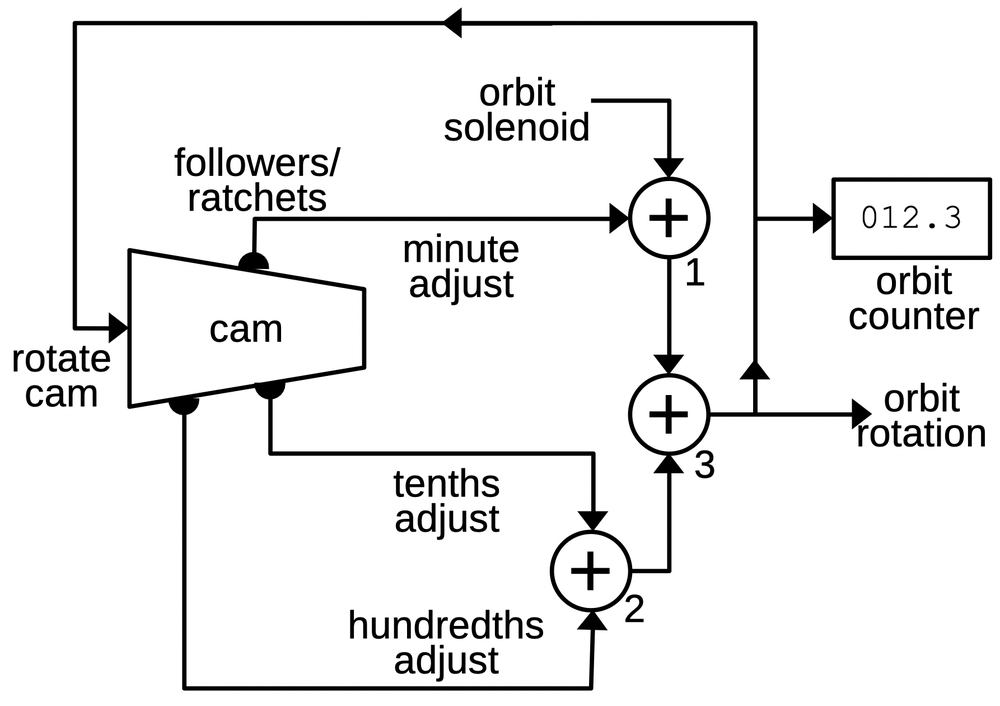A cam-based system adjusts the orbital speed using three differential gear assemblies.

The three adjustment signals are scaled by gear ratios to provide the appropriate contribution to the rotation. As shown above, the adjustments are added to the solenoid output by three differentials to generate the orbit rotation signal, output from differential 3.8 This signal also drives the odometer-like orbit counter on the front of the Globus. The diagram below shows how the components are arranged, as viewed from the back.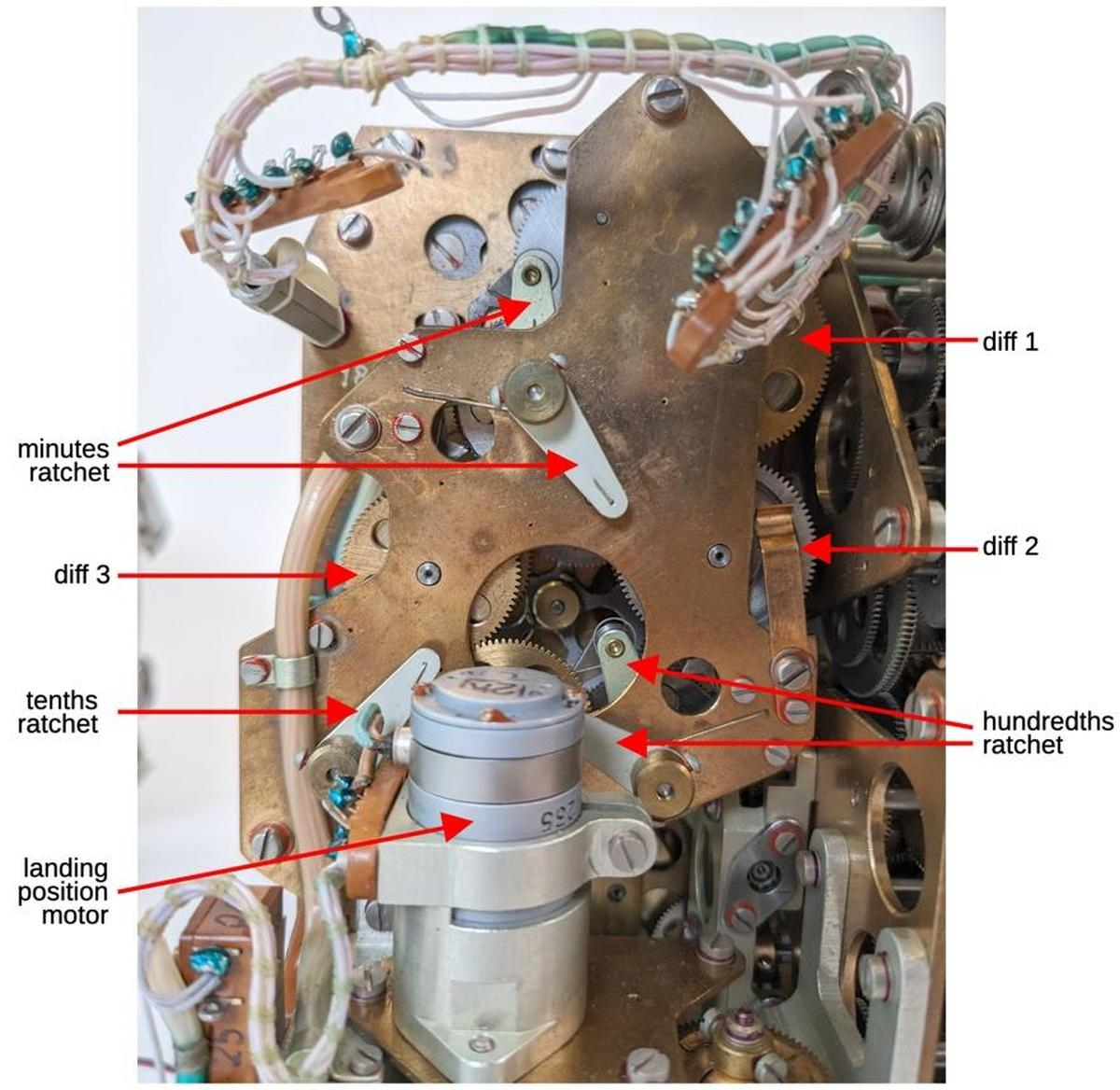A back view of the Globus showing the orbit components.

## Displaying the orbit rotation

Since the Globus doesn't have any external position input such as inertial guidance, it must be initialized by the cosmonauts. A knob on the front of the Globus provides manual adjustment of the orbital position. Differential 4 adds the knob signal to the orbit output discussed above.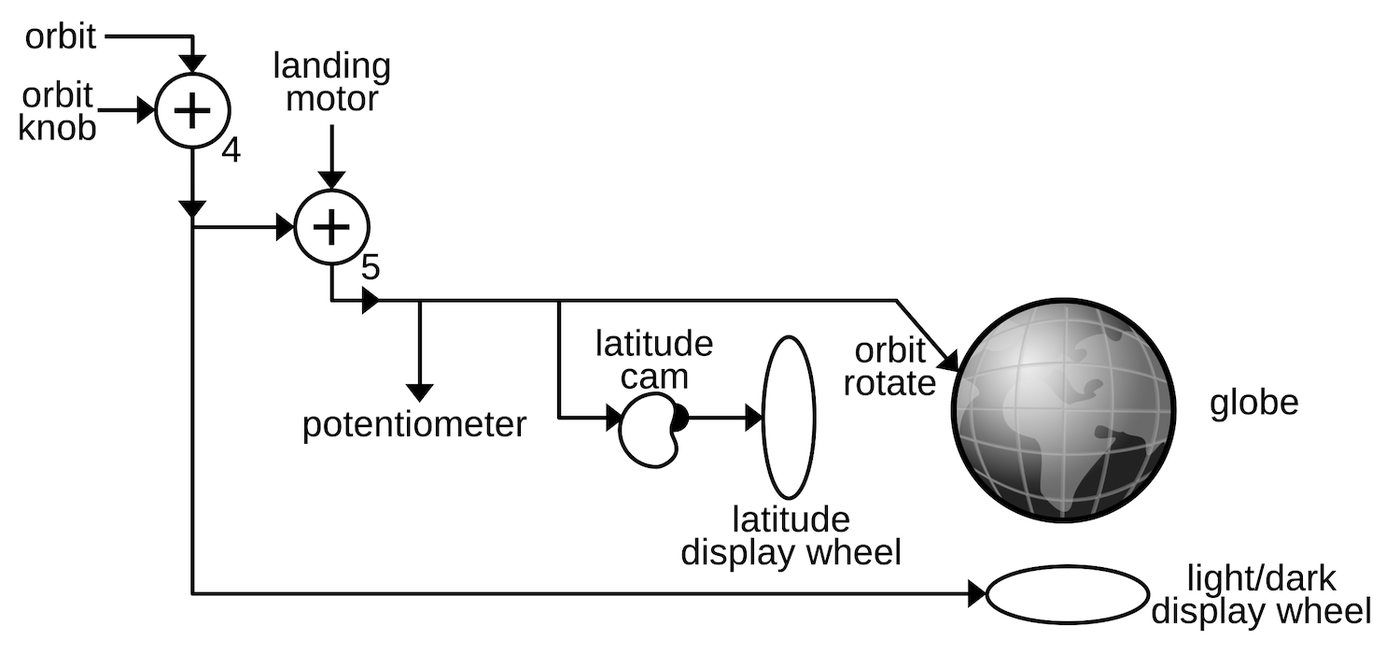The orbit controls drive the globe's motion.

The Globus has a "landing point" mode where the globe is rapidly rotated through a fraction of an orbit to indicate where the spacecraft would land if the retro-rockets were fired. Turning the mode switch caused the globe to rotate until the landing position was under the crosshairs and the cosmonauts could evaluate the suitability of this landing site. This mode is implemented with a landing position motor that provides the rapid rotation. This motor also rotates the globe back to the orbital position. The motor is driven through an electronics board with relays and a transistor, controlled by limit switches. I discussed the electronics in a previous post so I won't go into more details here. The landing position motor feeds into the orbit signal through differential 5, producing the final orbit signal.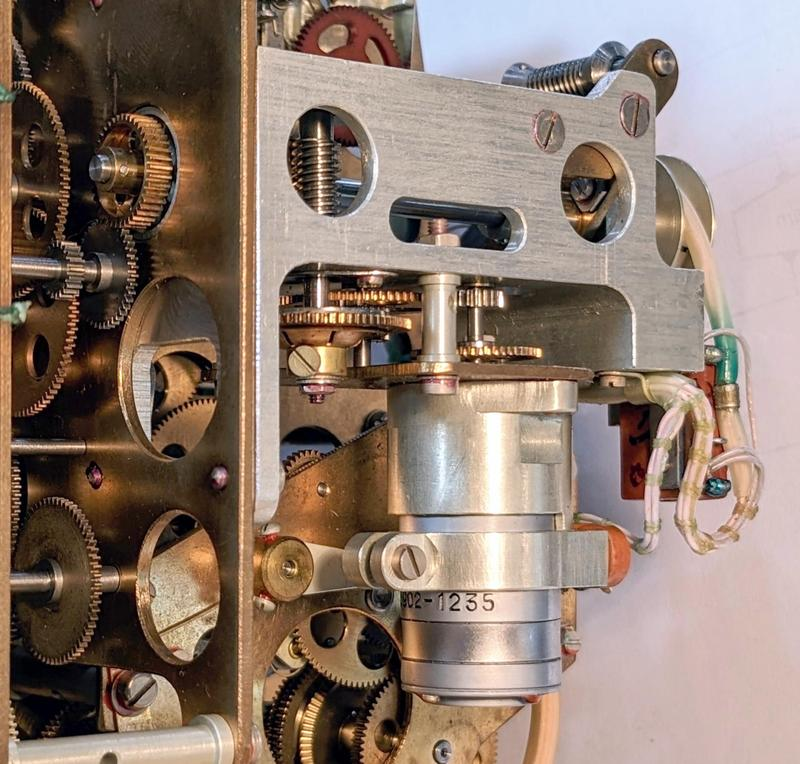The landing position motor and its associated gearing. The motor speed is geared down and then fed through a worm gear (upper center).

The orbit signal from differential 5 is used in several ways. Most importantly, the orbit signal provides the end-over-end rotation of the globe to indicate the spacecraft's travel in orbit. As discussed earlier, this is accomplished by rotating the globe's metal frame around the horizontal axis. The orbital signal also rotates a potentiometer to provide an electrical indication of the orbital position to other spacecraft systems.

Docking a spacecraft is a tricky endeavor, best performed in daylight, so it is useful to know how much time remains until the spacecraft enters the Earth's shadow. The light/shadow dial under the globe provides this information. This display consists of two nested wheels. The outer wheel is white and has two quarters removed. Through these gaps, the partially-black inner wheel is exposed, which can be adjusted to show 0% to 50% dark. This display is rotated by the orbital signal, turning half a revolution per orbit. As the spacecraft orbits, this dial shows the light/shadow transition and the time to the transistion.9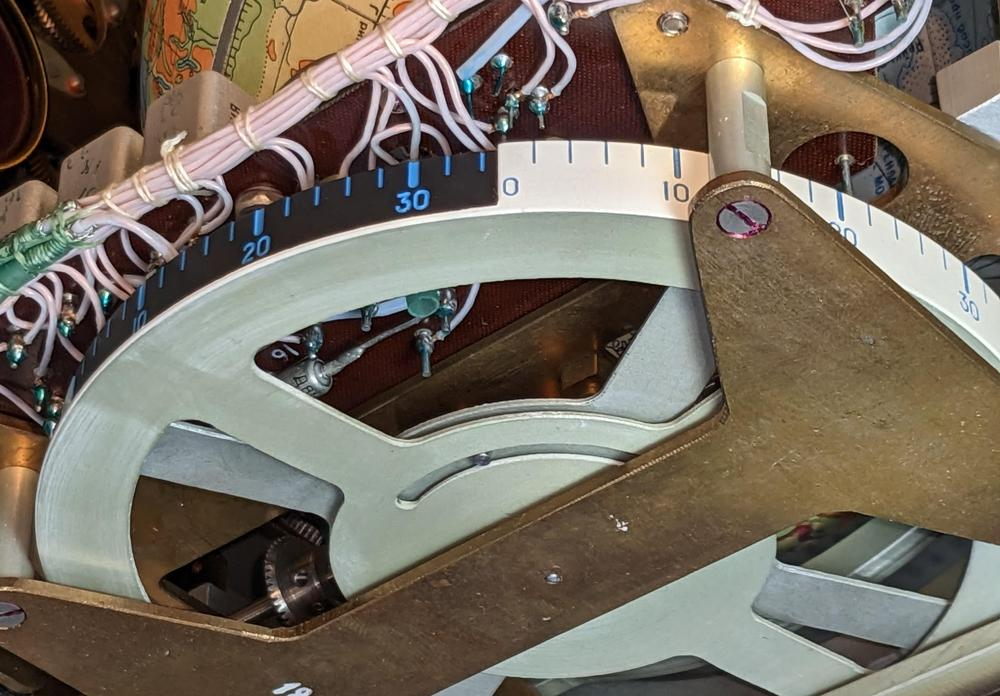The light/shadow indicator, viewed from the underside of the Globus. The shadow indicator has been set to 35% shadow. Near the hub, a pin restricts motion of the inner wheel relative to the outer wheel.

You might expect the orbit to be in the dark 50% of the time, but because the spacecraft is about 200 km above the Earth's surface, it will sometimes be illuminated when the surface of the Earth underneath is dark.10 In the ground track below, the dotted part of the track is where the spacecraft is in the Earth's shadow; this is considerably less than 50%. Also note that the end of the orbit doesn't match up with the beginning, due to the Earth's rotation during the orbit.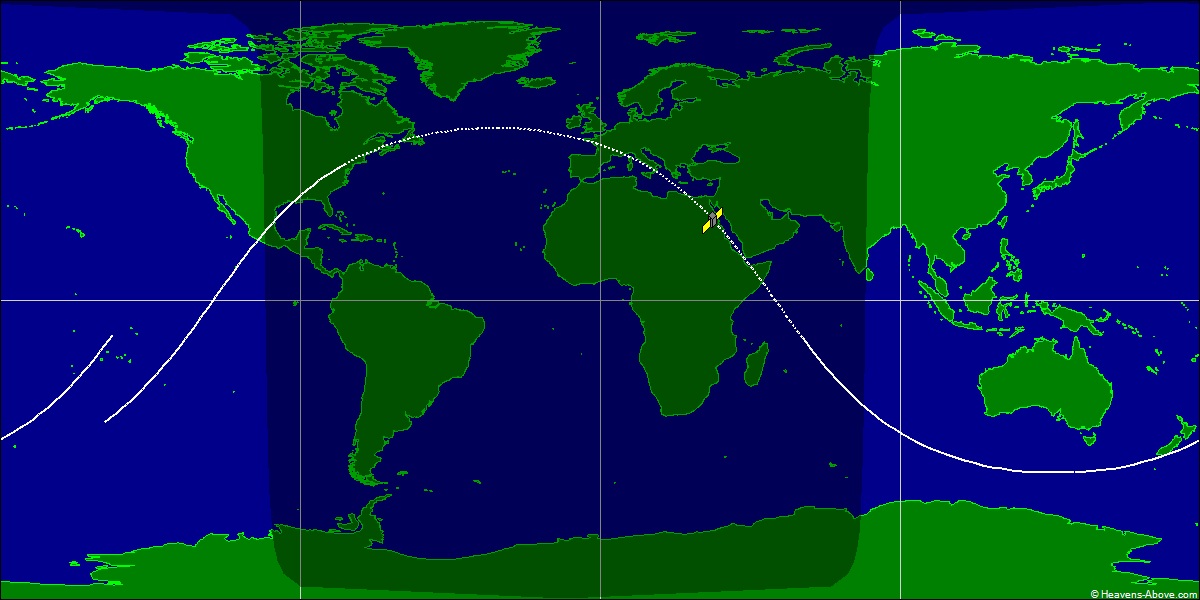Ground track of an Apollo-Soyuz Test Project orbit, corresponding to this Globus. Image courtesy of heavens-above.com.

## The latitude indicator

The latitude indicator to the left of the globe shows the spacecraft's latitude. The map above shows how the latitude oscillates between 51.8°N and 51.8°S, corresponding to the launch inclination angle. Even though the path around the globe is a straight (circular) line, the orbit appears roughly sinusoidal when projected onto the map.11 The exact latitude is a surprisingly complicated function of the orbital position.12 This function is implemented by a cam that is attached to the globe. The varying radius of the cam corresponds to the function. A follower tracks the profile of the cam and rotates the latitude display wheel accordingly, providing the non-linear motion.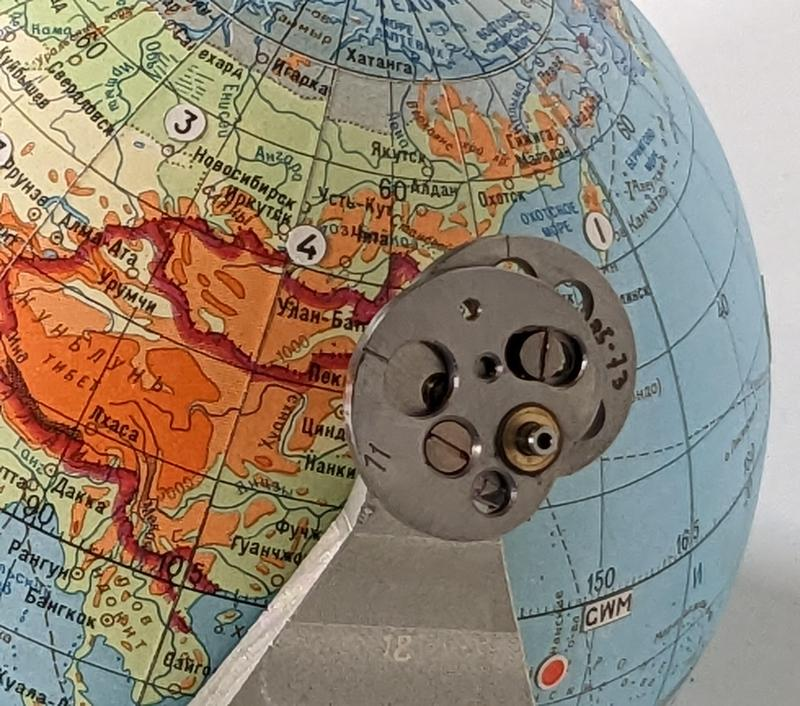A cam is attached to the globe and rotates with the globe.

## The Earth's rotation

The second motion of the globe is the Earth's daily rotation around its axis, which I'll call the Earth rotation. The Earth rotation is fed into the globe through the outer part of a concentric shaft, while the orbital rotation is provided through the inner shaft. The Earth rotation is transferred through three gears to the equatorial frame, where an internal mechanism rotates the hemispheres. There's a complication, though: if the globe's orbital shaft turns while the Earth rotation shaft remains stationary, the frame will rotate, causing the gears to turn and the hemispheres to rotate. In other words, keeping the hemispheres stationary requires the Earth shaft to rotate with the orbit shaft.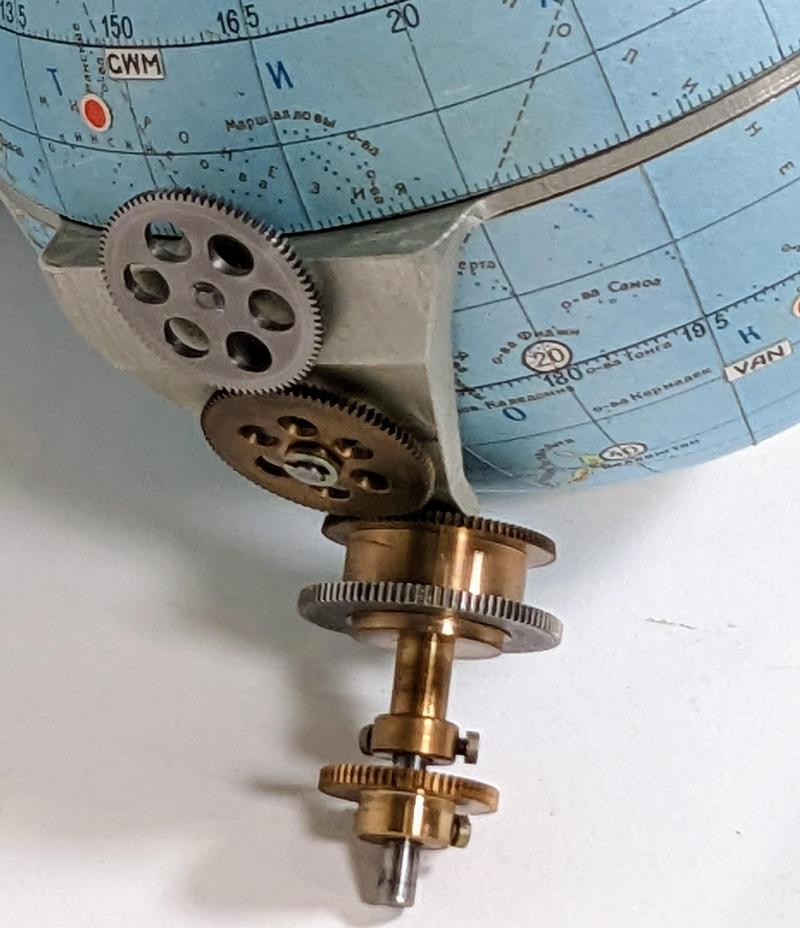A closeup of the gear mechanisms that drive the Globus, showing the concentric shafts that control the two rotations.

The Globus solves this problem by adding the orbit rotation to the Earth rotation, as shown in the diagram below, using differentials 7 and 8. Differential 8 adds the normal orbit rotation, while differential 7 adds the orbit rotation due to the landing motor.14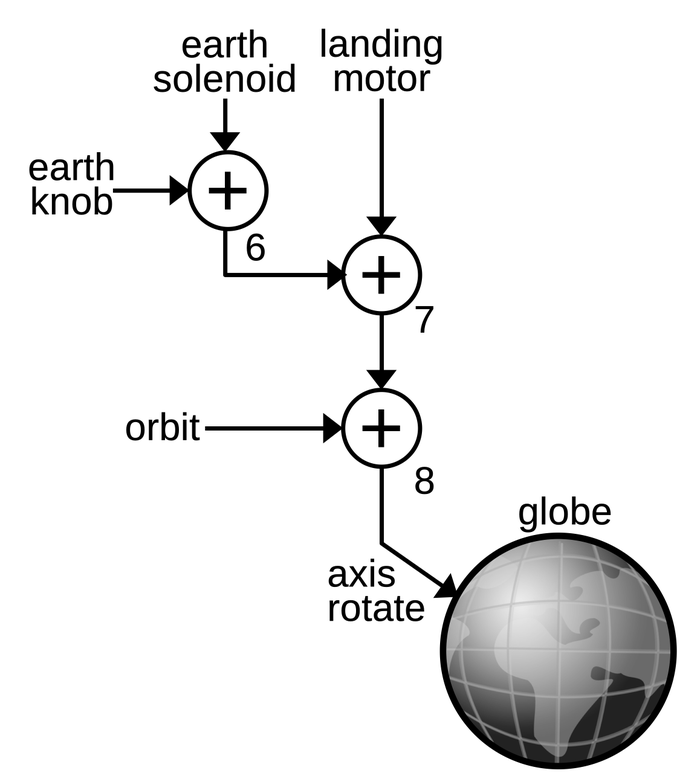The mechanism to compute the Earth's rotation around its axis.

The Earth motion is generated by a second solenoid (below) that is driven with one pulse per second.13 This motion is simpler than the orbit motion because it has a fixed rate. The "Earth" knob on the front of the Globus permits manual rotation around the Earth's axis. This signal is combined with the solenoid signal by differential 6. The sum from the three differentials is fed into the globe, rotating the hemispheres around their axis.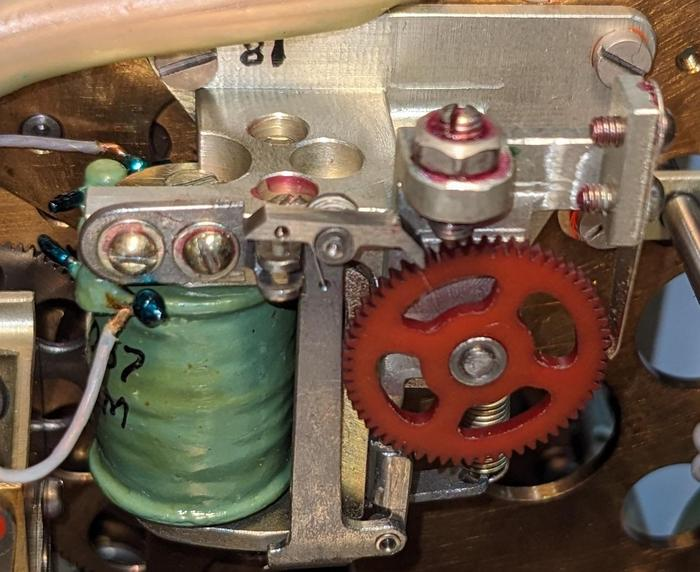This solenoid, ratchet, and gear on the underside of the Globus drive the Earth rotation.

The solenoid and differentials are visible from the underside of the Globus. The diagram below labels these components as well as other important components.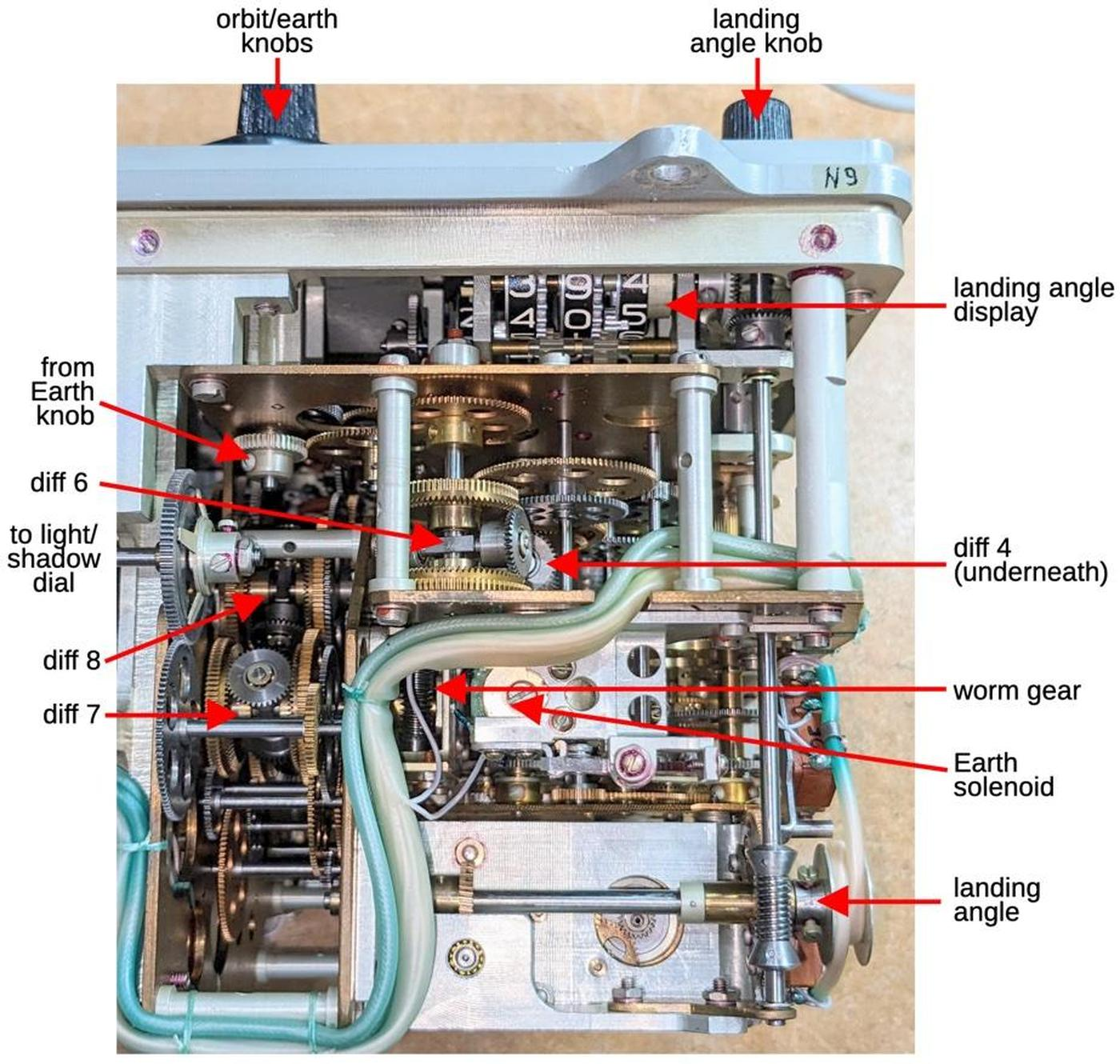The underside of the Globus.

## The longitude display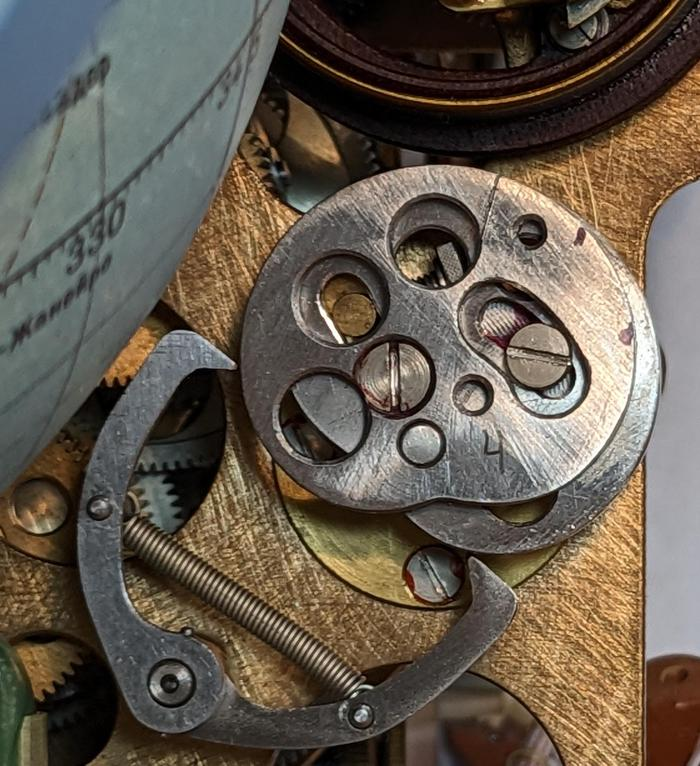The longitude cam and the followers that track its radius.

The longitude display is more complicated than the latitude display because it depends on both the Earth rotation and the orbit rotation. Unlike the latitude, the longitude doesn't oscillate but increases. The longitude increases by 360° every orbit according to a complicated formula describing the projection of the orbit onto the globe. Most of the time, the increase is small, but when crossing near the poles, the longitude changes rapidly. The Earth's rotation provides a smaller but steady negative change to the longitude.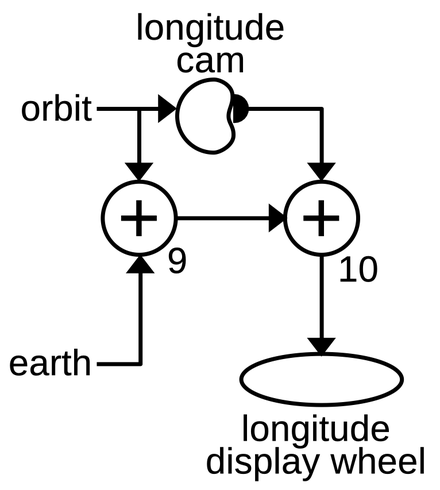The computation of the longitude.

The diagram above shows how the longitude is computed by combining the Earth rotation with the orbit rotation. Differential 9 adds the linear effect of the orbit on longitude (360° per orbit) and subtracts the effect of the Earth's rotation (360° per day). The nonlinear effect of the orbit is computed by a cam that is rotated by the orbit signal. The shape of the cam is picked up and fed into differential 10, computing the longitude that is displayed on the dial. The differentials, cam, and dial are visible from the back of the Globus (below).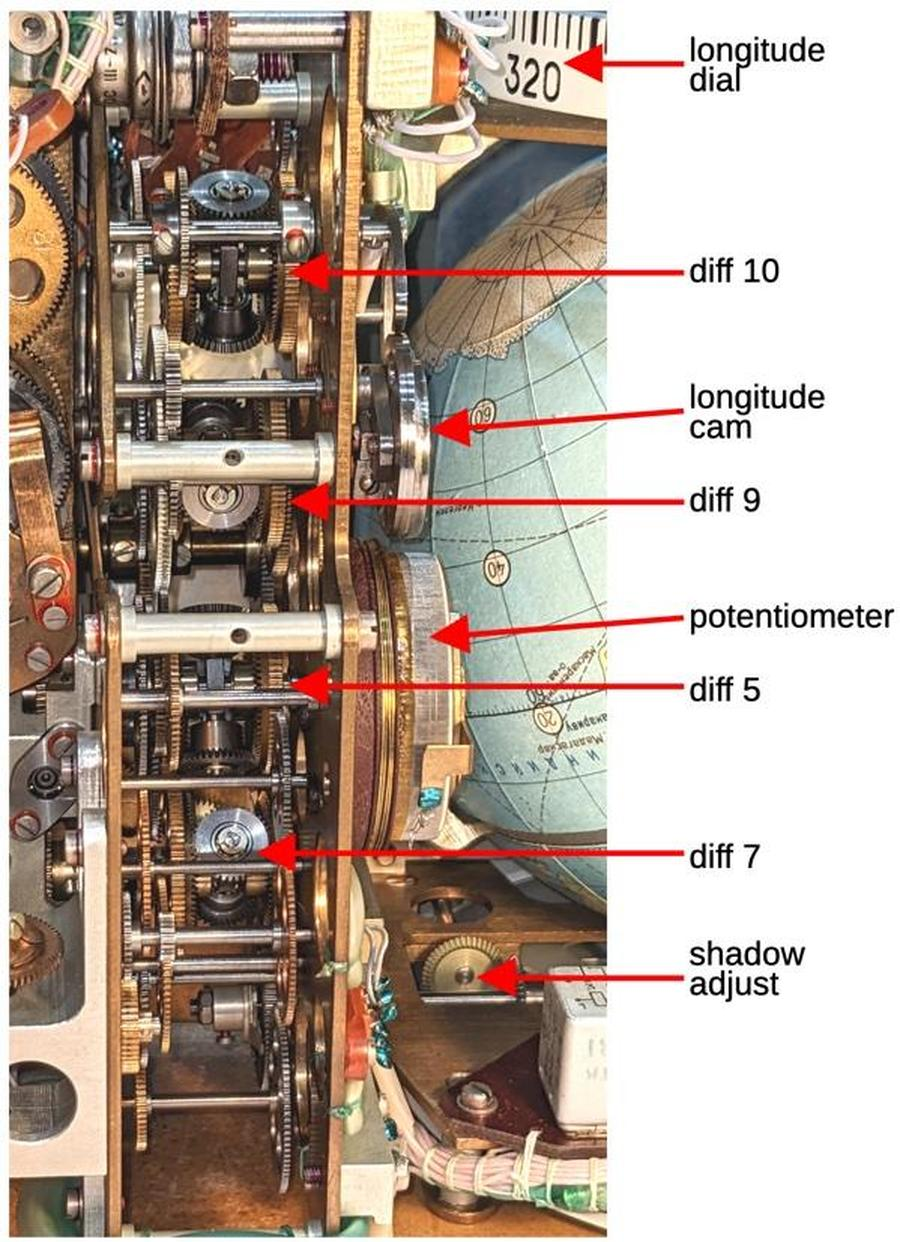A closeup of the differentials from the back of the Globus.

The time-lapse video below demonstrates the behavior of the rotating displays. The latitude display on the left oscillates between 51.8°N and 51.8°S. The longitude display at the top advances at a changing rate. Near the equator, it advances slowly, while it accelerates near the poles. The light/shadow display at the bottom rotates at a constant speed, completing half a revolution (one light/shadow cycle) per orbit.

## Conclusions

The Globus INK is a remarkable piece of machinery, an analog computer that calculates orbits through an intricate system of gears, cams, and differentials. It provided astronauts with a high-resolution, full-color display of the spacecraft's position, way beyond what an electronic space computer could provide in the 1960s.

The drawback of the Globus is that its functionality is limited. Its parameters must be manually configured: the spacecraft's starting position, the orbital speed, the light/shadow regions, and the landing angle. It doesn't take any external guidance inputs, such as an IMU (inertial measurement unit), so it's not particularly accurate. Finally, it only supports a circular orbit at a fixed angle. While a more modern digital display lacks the physical charm of a rotating globe, the digital solution provides much more capability.

I recently wrote blog posts providing a Globus overview and the Globus electronics. Follow me on Twitter @kenshirriff or RSS for updates. I've also started experimenting with Mastodon recently as @[email protected]. Many thanks to Marcel for providing the Globus. I worked on this with CuriousMarc, so check out his Globus videos.

## Notes and references

1. In Russian, the name for the device is "Индикатор Навигационный Космический" abbreviated as ИНК (INK). This translates to "space navigation indicator." but I'll use the more descriptive nickname "Globus" (i.e. globe). The Globus has a long history, back to the beginnings of Soviet crewed spaceflight. The first version was simpler and had the Russian acronym ИМП (IMP). Development of the IMP started in 1960 for the Vostok (1961) and Voshod (1964) spaceflights. The more complex INK model (described in this blog post) was created for the Soyuz flights, starting in 1967. The landing position feature is the main improvement of the INK model. The Soyuz-TMA (2002) upgraded to the Neptun-ME system which used digital display screens and abandoned the Globus.

2. According to this document, one revolution of the globe relative to the axis of daily rotation occurs in a time equal to a sidereal day, taking into account the precession of the orbit relative to the earth's axis, caused by the asymmetry of the Earth's gravitational field. (A sidereal day is approximately 4 minutes shorter than a regular 24-hour day. The difference is that the sidereal day is relative to the fixed stars, rather than relative to the Sun.)

3. To see how the angle between the poles and the globe's rotation results in the desired orbital inclination, consider two limit cases. First, suppose the angle between is 90°. In this case, the globe is "straight" with the equator horizontal. Rotating the globe along the horizontal axis, flipping the poles end-over-end, will cause the crosshair to trace a polar orbit, giving the expected inclination of 90°. On the other hand, suppose the angle is 0°. In this case, the globe is "sideways" with the equator vertical. Rotating the globe will cause the crosshair to remain over the equator, corresponding to an equatorial orbit with 0° inclination.

4. There is a bit of ambiguity when describing the gear motions. If the end gears are rotating upwards when viewed from the front, the gears are both rotating clockwise when viewed from the right, so I'm referring to them as rotating in the same direction. But if you view each gear from its own side, the gear on the left is turning counterclockwise, so from that perspective they are turning in opposite directions.

5. The solenoids are important since they provide all the energy to drive the globe. One of the problems with gear-driven analog computers is that each gear and shaft has a bit of friction and loses a bit of torque, and there is nothing to amplify the signal along the way. Thus, the 27-volt solenoids need to provide enough force to run the entire system.

6. The orbital time can be adjusted between 86.85 minutes and 96.85 minutes according to this detailed page that describes the Globus in Russian.

7. The Globus is manufactured for a particular orbital inclination, in this case 51.8°. The Globus assumes a circular orbit and does not account for any variations. The Globus does not account for any maneuvering in orbit.

8. The outputs from the orbit cam are fed into the overall orbit rotation, which drives the orbit cam. This may seem like an "infinite loop" since the outputs from the cam turn the cam itself. However, the outputs from the cam are a small part of the overall orbit rotation, so the feedback dies off.

9. The scales on the light/shadow display are a bit confusing. The inner scale (blue) is measured in percentage of an orbit, up to 100%. The fixed outer scale (red) measures minutes, indicating how many minutes until the spacecraft enters or leaves shadow. The spacecraft completes 100% of an orbit in about 90 minutes, so the scales almost, but not quite, line up. The wheel is driven by the orbit mechanism and turns half a revolution per orbit.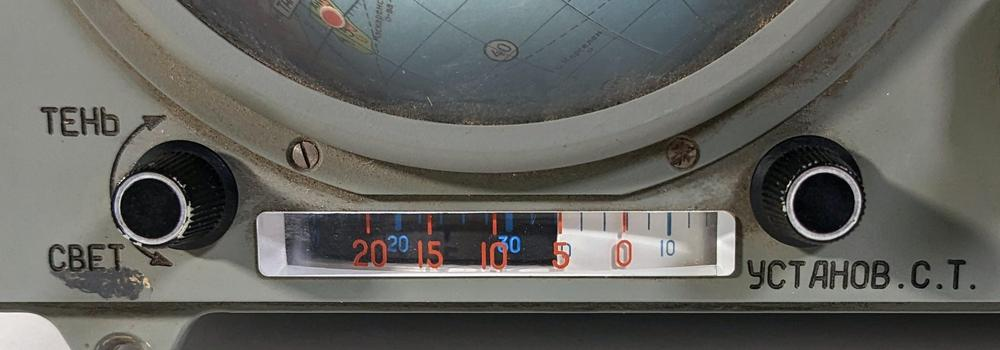The light and shadow indicator is controlled by two knobs.

10. The Internation Space Station illustrates how an orbiting spacecraft is illuminated more than 50% of the time due to its height. You can often see the ISS illuminated in the nighttime sky close to sunset and sunrise (link).

11. The ground track on the map is roughly, but not exactly, sinusoidal. As the orbit swings further from the equator, the track deviates more from a pure sinusoid. The shape will depend, of course, on the rectangular map projection. For more information, see this StackExcahnge post

12. To get an idea of how the latitude and longitude behave, consider a polar orbit with 90° angle of inclination, one that goes up a line of longitude, crosses the North Pole, and goes down the opposite line of latitude. Now, shift the orbit away from the poles a bit, but keeping a great circle. The spacecraft will go up, nearly along a constant line of longitude, with the latitude increasing steadily. As the spacecraft reaches the peak of its orbit near the North Pole, it will fall a bit short of the Pole but will still rapidly cross over to the other side. During this phase, the spacecraft rapidly crosses many lines of longitude (which are close together near the Pole) until it reaches the opposite line of longitude. Meanwhile, the latitude stops increasing short of 90° and then starts dropping. On the other side, the process repeats, with the longitude nearly constant while the latitude drops relatively constantly.

The latitude and longitude are generated by complicated trigonometric functions. The latitude is given by arcsin(sin i * sin (2πt/T)), while the longitude is given by λ = arctan (cos i * tan(2πt/T)) + Ωt + λ0, where t is the spaceship's flight time starting at the equator, i is the angle of inclination (51.8°), T is the orbital period, Ω is the angular velocity of the Earth's rotation, and λ0 is the longitude of the ascending node.

13. An important function of the gears is to scale the rotations as needed by using different gear ratios. For the most part, I'm ignoring the gear ratios, but the Earth rotation gearing is interesting. The gear driven by the solenoid has 60 teeth, so it rotates exactly once per minute. This gear drives a shaft with a very small gear on the other end with 15 teeth. This gear meshes with a much larger gear with approximately 75 teeth, which will thus rotate once every 5 minutes. The other end of that shaft has a gear with approximately 15 teeth, meshed with a large gear with approximately 90 teeth. This divides the rate by 6, yielding a rotation every 30 minutes. The sequence of gears and shafts continues, until the rotation is reduced to once per day. (The tooth counts are approximate because the gears are partially obstructed inside the Globus, making counting difficult.)

14. There's a potential simplification when canceling out the orbital shaft rotation from the Earth rotation. If the orbit motion was taken from differential 5 instead of differential 4, the landing motor effect would get added automatically, eliminating the need for differential 7. I think the landing motor motion was added separately so the mechanism could account for the Earth's rotation during the landing descent.

### Reverse-engineering the multiplication algorithm in the Intel 8086 processor

While programmers today take multiplication for granted, most microprocessors in the 1970s could only add and subtract — multiplication required a slow and tedious loop implemented in assembly code.1 One of the nice features of the Intel 8086 processor (1978) was that it provided machine instructions for multiplication,2 able to multiply 8-bit or 16-bit numbers with a single instruction. Internally, the 8086 still performed a loop, but the loop was implemented in microcode: faster and transparent to the programmer. Even so, multiplication was a slow operation, about 24 to 30 times slower than addition.

In this blog post, I explain the multiplication process inside the 8086, analyze the microcode that it used, and discuss the hardware circuitry that helped it out.3 My analysis is based on reverse-engineering the 8086 from die photos. The die photo below shows the chip under a microscope. I've labeled the key functional blocks; the ones that are important to this post are darker. At the left, the ALU (Arithmetic/Logic Unit) performs the arithmetic operations at the heart of multiplication: addition and shifts. Multiplication also uses a few other hardware features: the X register, the F1 flag, and a loop counter. The microcode ROM at the lower right controls the process.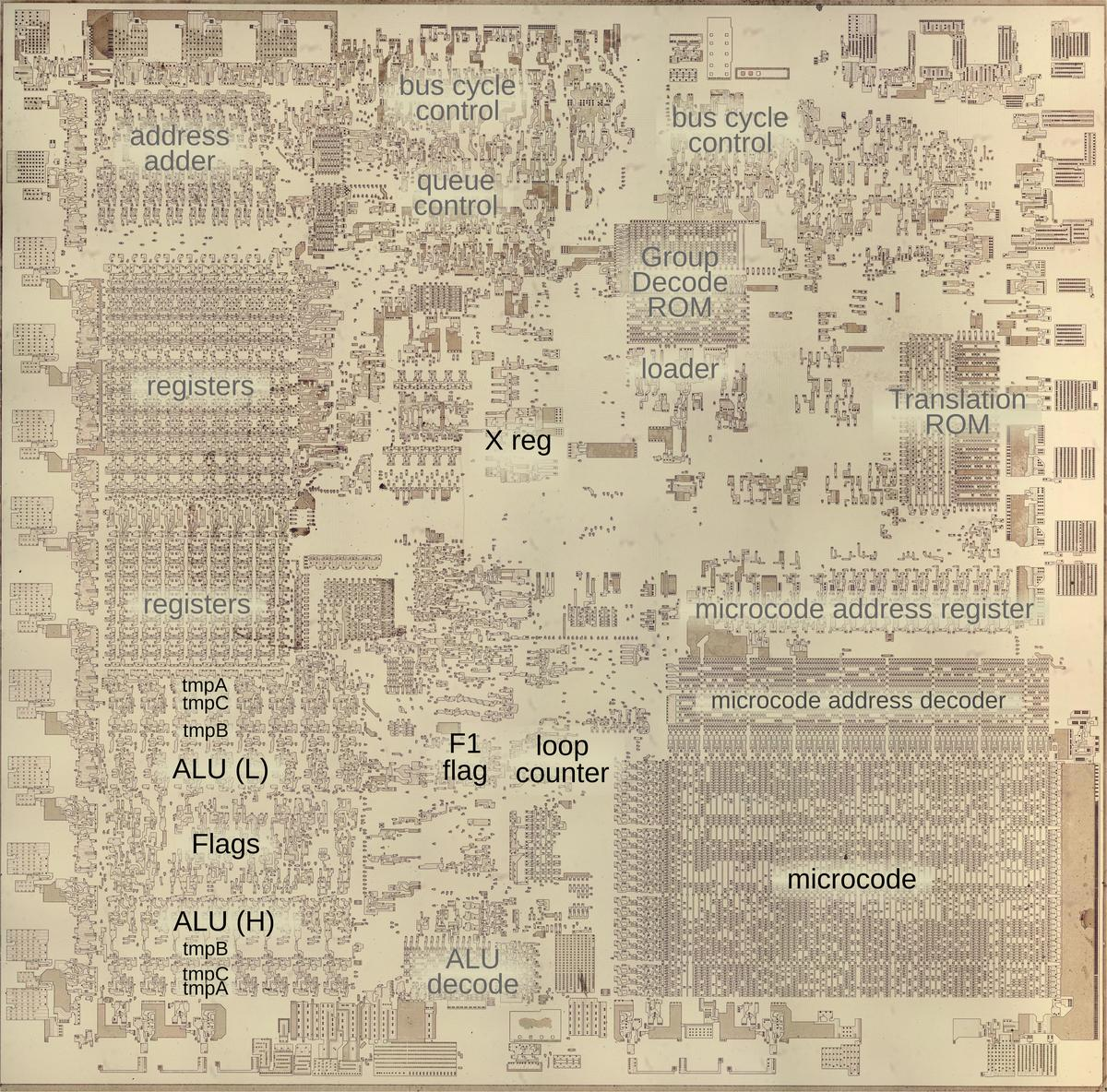The 8086 die under a microscope, with main functional blocks labeled. This photo shows the chip with the metal and polysilicon removed, revealing the silicon underneath. Click on this image (or any other) for a larger version.

## Microcode

The multiplication routines in the 8086 are implemented in microcode. Most people think of machine instructions as the basic steps that a computer performs. However, many processors (including the 8086) have another layer of software underneath: microcode. With microcode, instead of building the control circuitry from complex logic gates, the control logic is largely replaced with code. To execute a machine instruction, the computer internally executes several simpler micro-instructions, specified by the microcode. This is especially useful for a machine instruction such as multiplication, which requires many steps in a loop.

A micro-instruction in the 8086 is encoded into 21 bits as shown below. Every micro-instruction has a move from a source register to a destination register, each specified with 5 bits. The meaning of the remaining bits depends on the type field and can be anything from an ALU operation to a memory read or write to a change of microcode control flow. Thus, an 8086 micro-instruction typically does two things in parallel: the move and the action. For more about 8086 microcode, see my microcode blog post.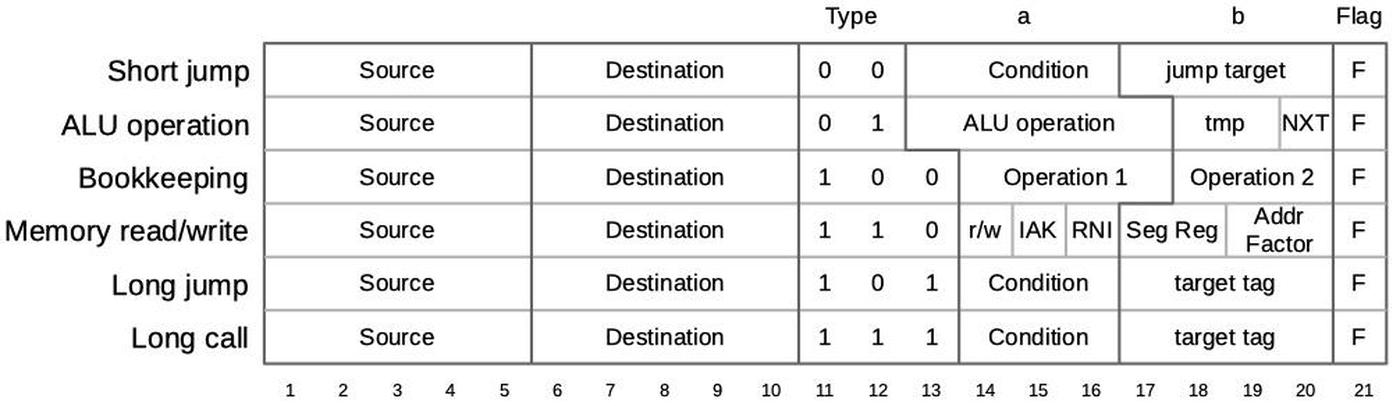The encoding of a micro-instruction into 21 bits. Based on NEC v. Intel: Will Hardware Be Drawn into the Black Hole of Copyright?

The behavior of an ALU micro-operation is important for multiplication. The ALU has three temporary registers that are invisible to the programmer: tmpA, tmpB, and tmpC. An ALU operation takes its first argument from any temporary register, while the second argument always comes from tmpB. An ALU operation requires two micro-instructions. The first micro-instruction specifies the ALU operation and source register, configuring the ALU. For instance, `ADD tmpA` to add tmpA to the default tmpB. In the next micro-instruction (or a later one), the ALU result can be accessed through the `Σ` register and moved to another register.

Before I get into the microcode routines, I should explain two ALU operations that play a central role in multiplication: `LRCY` and `RRCY`, Left Rotate through Carry and Right Rotate through Carry. (These correspond to the `RCL` and `RCR` machine instructions, which rotate through carry left or right.) These operations shift the bits in a 16-bit word, similar to the `<<` and `>>` bit-shift operations in high-level languages, but with an additional feature. Instead of discarding the bit on the end, that bit is moved into the carry flag (`CF`). Meanwhile, the bit formerly in the carry flag moves into the word. You can think of this as rotating the bits while treating the carry flag as a 17th bit of the word.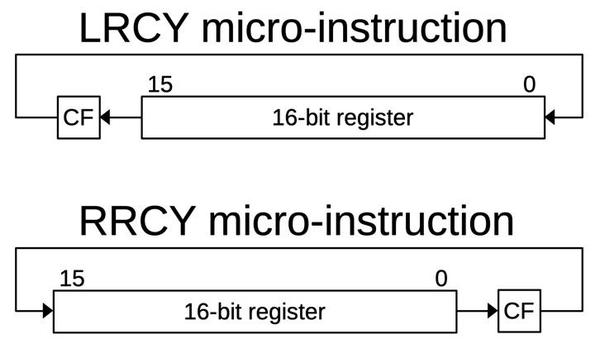The left rotate through carry and right rotate through carry micro-instructions.

These shifts perform an important part of the multiplication process since shifting can be viewed as multiplying by two. `LRCY` also provides a convenient way to move the most-significant bit to the carry flag, where it can be tested for a conditional jump. (This is important because the top bit is used as the sign bit.) Similarly, `RRCY` provides access to the least significant bit, very important for the multiplication process. Another important property is that performing `RRCY` on an upper word and then `RRCY` on a lower word will perform a 32-bit shift, since the low bit of the upper word will be moved into the high bit of the lower word via the carry bit.

## Binary multiplication

The shift-and-add method of multiplication (below) is similar to grade-school long multiplication, except it uses binary instead of decimal. In each row, the multiplicand is multiplied by one digit of the multiplier. (The multiplicand is the value that gets repeatedly added, and the multiplier controls how many times it gets added.) Successive rows are shifted left one digit. At the bottom, the rows are added together to yield the product. The example below shows how 6×5 is calculated in binary using long multiplication.

0110
×0101
0110
0000
0110
0000
00011110

Binary long multiplication is much simpler than decimal multiplication: at each step, you're multiplying by 0 or 1. Thus, each row is either zero or the multiplicand appropriately shifted (0110 in this case). (Unlike decimal long multiplication, you don't need to know the multiplication table.) This simplifies the hardware implementation, since each step either adds the multiplicand or doesn't. In other words, each step tests a bit of the multiplier, starting with the low bit, to determine if an add should take place or not. This bit can be obtained by shifting the multiplier one bit to the right each step.

Although the diagram above shows the sum at the end, a real implementation performs the addition at each step of the loop, keeping a running total. Moreover, in the 8086, instead of shifting the multiplicand to the left during each step, the sum shifts to the right. (The result is the same but it makes the implementation easier.) Thus, multiplying 6×5 goes through the steps below.

0101
×0110
00000
001010
0011110
00011110

Why would you shift the result to the right? There's a clever reason for this. Suppose you're multiplying two 16-bit numbers, which yields a 32-bit result. That requires four 16-bit words of storage if you use the straightforward approach. But if you look more closely, the first sum fits into 16 bits, and then you need one more bit at each step. Meanwhile, you're "using up" one bit of the multiplier at each step. So if you squeeze the sum and the multiplier together, you can fit them into two words. Shifting right accomplishes this, as the diagram below illustrates for `0xffff`×`0xf00f`. The sum (blue) starts in a 16-bit register called `tmpA` while the multiplier (green) is stored in the 16-bit `tmpB` register. In each step, they are both shifted right, so the sum gains one bit and the multiplier loses one bit. By the end, the sum takes up all 32 bits, split across both registers.

sum (tmpA)multiplier (tmpC)
00000000000000001111000000001111
01111111111111111111100000000111
10111111111111110111110000000011
11011111111111110011111000000001
11101111111111110001111100000000
01110111111111111000111110000000
00111011111111111100011111000000
00011101111111111110001111100000
00001110111111111111000111110000
00000111011111111111100011111000
00000011101111111111110001111100
00000001110111111111111000111110
00000000111011111111111100011111
10000000011101110111111110001111
11000000001110110011111111000111
11100000000111010001111111100011
11110000000011100000111111110001

## The multiplication microcode

The 8086 has four multiply instructions to handle signed and unsigned multiplication of byte and word operands. These machine instructions are implemented in microcode. I'll start by describing the unsigned word multiplication, which multiplies two 16-bit values and produces a 32-bit result. The source word is provided by either a register or memory. It is multiplied by `AX`, the accumulator register. The 32-bit result is returned in the `DX` and `AX` registers.

The microcode below is the main routine for word multiplication, both signed and unsigned. Each micro-instruction specifies a register move on the left, and an action on the right. The moves transfer words between the visible registers and the ALU's temporary registers, while the actions are mostly subroutine calls to other micro-routines.

```  move        action
AX → tmpC   LRCY tmpC        iMUL rmw:
M → tmpB    CALL X0 PREIMUL   called for signed multiplication
CALL CORX         the core routine
CALL F1 NEGATE    called for negative result
CALL X0 IMULCOF   called for signed multiplication
tmpC → AX   JMPS X0 7
CALL MULCOF       called for unsigned multiplication
tmpA → DX   RNI
```

The microcode starts by moving one argument `AX` into the ALU's temporary C register and setting up the ALU to perform a Left Rotate through Carry on this register, in order to access the sign bit. Next, it moves the second argument `M` into the temporary B register; `M` references the register or memory specified in the second byte of the instruction, the "ModR/M" byte. For a signed multiply instruction, the `PREIMUL` micro-subroutine is called, but I'll skip that for now. (The `X0` condition tests bit 3 of the instruction, which in this case distinguishes `MUL` from `IMUL`.) Next, the `CORX` subroutine is called, which is the heart of the multiplication.4 If the result needs to be negated (indicated by the `F1` condition), the `NEGATE` micro-subroutine is called. For signed multiplication, `IMULCOF` is then called to set the carry and overflow flags, while `MULCOF` is called for unsigned multiplication. Meanwhile, the result bytes are moved from the temporary C and temporary registers to the `AX` and `DX` registers. Finally, `RNI` runs the next machine instruction, ending the microcode routine.

### `CORX`

The heart of the multiplication code is the `CORX` routine, which performs the multiplication loop, computing the product through shifts and adds. The first two lines set up the loop, initializing the sum (tmpA) to 0. The number of loops is controlled by a special-purpose loop counter. The `MAXC` micro-instruction initializes the counter to 7 or 15, for a byte or word multiply respectively. The first shift of tmpC is performed, putting the low bit into the carry flag.

The loop body performs the shift-and-add step. It tests the carry flag, the low bit of the multiplicand. It skips over the `ADD` if there is no carry (`NCY`). Otherwise, tmpB is added to tmpA. (As tmpA gets shifted to the right, tmpB gets added to higher and higher positions in the result.) The tmpA and tmpC registers are rotated right. This also puts the next bit of the multiplicand into the carry flag for the next cycle. The microcode jumps to the top of the loop if the counter is not zero (`NCZ`). Otherwise, the subroutine returns with the result in tmpA and tmpC.

```ZERO → tmpA  RRCY tmpC   CORX: initialize right rotate
Σ → tmpC     MAXC          get rotate result, initialize counter to max value
JMPS NCY 8  5: top of loop
Σ → tmpA               F  sum to tmpA, update flags to get carry
RRCY tmpA   8: 32-bit shift of tmpA/tmpC
Σ → tmpA     RRCY tmpC
Σ → tmpC     JMPS NCZ 5   loop to 5 if counter is not 0
RTN
```

### `MULCOF`

The last subroutine is `MULCOF`, which configures the carry and overflow flags. The 8086 uses the rule that if the upper half of the result is nonzero, the carry and overflow flags are set, otherwise they are cleared. The first two lines pass tmpA (the upper half of the result) through the ALU to set the zero flag for the conditional jump. As a side-effect, the other status flags will get set but these values are "undefined" in the documentation.6 If the test is nonzero, the carry and overflow flags are set (`SCOF`), otherwise they are cleared (`CCOF`).5 The `SCOF` and `CCOF` micro-operations were implemented solely for used by multiplication, illustrating how microcode can be designed around specific needs.

```             PASS tmpA  MULCOF: pass tmpA through to test if zero
Σ → no dest  JMPS 12   F update flags

JMPS Z 8   12: jump if zero
SCOF RTN    otherwise set carry and overflow

CCOF RTN   8: clear carry and overflow
```

## 8-bit multiplication

The 8086 has separate instructions for 8-bit multiplication. The process for 8-bit multiplication is similar to 16-bit multiplication, except the values are half as long and the shift-and-add loop executes 8 times instead of 16. As shown below, the 8-bit sum starts in the low half of the temporary A register and is shifted left into tmpC. Meanwhile, the 8-bit multiplier starts in the low half of tmpC and is shifted out to the right. At the end, the result is split between tmpA and tmpC.

sum (tmpA)multiplier (tmpC)
00000000000000000000000001010101
00000000011111111000000000101010
00000000001111111100000000010101
00000000100111110110000000001010
00000000010011111011000000000101
00000000101001110101100000000010
00000000010100111010110000000001
00000000101010010101011000000000
00000000010101001010101100000000

The 8086 supports many instructions with byte and word versions, using 8-bit or 16-bit arguments. In most cases, the byte and word instructions use the same microcode, with the ALU and register hardware using bytes or words based on the instruction. However, the byte- and word-multiply instructions use different registers, requiring microcode changes. In particular, the multiplier is in `AL`, the low half of the accumulator. At the end, the 16-bit result is returned in `AX`, the full 16-bit accumulator; two micro-instructions assemble the result from tmpC and tmpA into the two bytes of the accumulator, 'AL' and 'AH' respectively. Apart from those changes, the microcode is the same as the word multiply microcode discussed earlier.

```AL → tmpC    LRCY tmpC         iMUL rmb:
M → tmpB     CALL X0 PREIMUL
CALL CORX
CALL F1 NEGATE
CALL X0 IMULCOF
tmpC → AL    JMPS X0 7
CALL MULCOF
tmpA → AH    RNI
```

## Signed multiplication

The 8086 (like most computers) represents signed numbers using a format called two's complement. While a regular byte holds a number from 0 to 255, a signed byte holds a number from -128 to 127. A negative number is formed by flipping all the bits (known as the one's complement) and then adding 1, yielding the two's complement value.7 For instance, +5 is `0x05` while -5 is `0xfb`. (Note that the top bit of a number is set for a negative number; this is the sign bit.) The nice thing about two's complement numbers is that the same addition and subtraction operations work on both signed and unsigned values. Unfortunately, this is not the case for signed multiplication, since signed and unsigned values yield different results due to sign extension.

The 8086 has separate multiplication instructions `IMUL` (Integer Multiply) to perform signed multiplication. The 8086 performs signed multiplication by converting the arguments to positive values, performing unsigned multiplication, and then negating the result if necessary. As shown above, signed and unsigned multiplication both use the same microcode, but the microcode conditionally calls some subroutines for signed multiplication. I will discuss those micro-subroutines below.

### `PREIMUL`

The first subroutine for signed multiplication is `PREIMUL`, performing preliminary operations for integer multiplication. It converts the two arguments, stored in tmpC and tmpB, to positive values. It keeps track of the signs using an internal flag called `F1`, toggling this flag for a negative argument. This conveniently handles the rule that two negatives make a positive since complementing the `F1` flag twice will clear it.

This microcode, below, illustrates the complexity of microcode and how micro-operations are carefully arranged to get the right values at the right time. The first micro-instruction performs one ALU operation and sets up a second operation. The calling code had set up the ALU to perform `LRCY tmpC`, so that's the result returned by Σ (and discarded). Performing a left rotate and discarding the result may seem pointless, but the important side-effect is that the top bit (i.e. the sign bit) ends up in the carry flag. The microcode does not have a conditional jump based on the sign, but has a conditional jump based on carry, so the point is to test if tmpC is negative. The first micro-instruction also sets up negation (`NEG tmpC`) for the next ALU operation.

```Σ → no dest  NEG tmpC   PREIMUL: set up negation of tmpC
JMPS NCY 7  jump if tmpC positive
Σ → tmpC     CF1         if negative, negate tmpC, flip F1

LRCY tmpB  7:
Σ → no dest  NEG tmpB    set up negation of tmpB
JMPS NCY 11 jump if tmpB positive
Σ → tmpB     CF1 RTN     if negative, negate tmpB, flip F1
RTN        11: return
```

For the remaining lines, if the carry is clear (`NCY`), the next two lines are skipped. Otherwise, the ALU result (`Σ`) is written to tmpC, making it positive, and the `F1` flag is complemented with `CF1`. (The second short jump (`JMPS`) may look unnecessary, but I reordered the code for clarity.) The second half of the microcode performs a similar test on tmpB. If tmpB is negative, it is negated and `F1` is toggled.

### `NEGATE`

The microcode below is called after computing the result, if the result needs to be made negative. Negation is harder than you might expect because the result is split between the tmpA and tmpC registers. The two's complement operation (`NEG`) is applied to the low word, while either 2's complement or one's complement (`COM1`) is applied to the upper word, depending on the carry for mathematical reasons.8 The code also toggles F1 and makes tmpB positive; I think this code is only useful for division, which also uses the `NEGATE` subroutine.

```             NEG tmpC   NEGATE: negate tmpC
Σ → tmpC     COM1 tmpA F maybe complement tmpA
JMPS CY 6
NEG tmpA    negate tmpA if there's no carry
Σ → tmpA     CF1        6: toggle F1 for some reason

LRCY tmpB  7: test sign of tmpB
Σ → no dest  NEG tmpB    maybe negate tmpB
JMPS NCY 11 skip if tmpB positive
Σ → tmpB     CF1 RTN     else negate tmpB, toggle F1
RTN        11: return
```

### `IMULCOF`

The `IMULCOF` routine is similar to `MULCOF`, but the calculation is a bit trickier for a signed result. This routine sets the carry and overflow flags if the upper half of the result is significant, that is, it is not just the sign extension of the lower half.9 In other words, the top byte is not significant if it duplicates the top bit (the sign bit) of the lower byte. The trick in the microcode is to add the top bit of the lower byte to the upper byte by putting it in the carry flag and performing an add with carry (`ADC`) of 0. If the result is 0, the upper byte is not significant, handling the positive and negative cases. (This also holds for words instead of bytes.)

```ZERO → tmpB  LRCY tmpC  IMULCOF: get top bit of tmpC
Σ → no dest  ADC tmpA    add to tmpA and 0 (tmpB)
Σ → no dest   F          update flags
JMPS Z 8   12: jump if zero result
SCOF RTN    otherwise set carry and overflow

CCOF RTN   8: clear carry and overflow
```

## The hardware for multiplication

For the most part, the 8086 uses the regular ALU addition and shifts for the multiplication algorithm. Some special hardware features provide assistance.

### Loop counter

The 8086 has a special 4-bit loop counter for multiplication. This counter starts at 7 for byte multiplication and 15 for word multiplication, based on the instruction. This loop counter allows the microcode to decrement the counter, test for the end, and perform a conditional branch in one micro-operation. The counter is implemented with four flip-flops, along with logic to compute the value after decrementing by one. The `MAXC` (Maximum Count) micro-instruction sets the counter to 7 or 15 for byte or word operations respectively. The `NCZ` (Not Counter Zero) micro-instruction has two actions. First, it performs a conditional jump if the counter is nonzero. Second, it decrements the counter.

### X register

The multiplication microcode uses an internal register called the `X` register to distinguish between the `MUL` and `IMUL` instructions. The `X` register is a 3-bit register that holds the ALU opcode, indicated by bits 5–3 of the instruction.10 Since the instruction is held in the Instruction Register, you might wonder why a separate register is required. The motivation is that some opcodes specify the type of ALU operation in the second byte of the instruction, the ModR/M byte, bits 5–3.11 Since the ALU operation is sometimes specified in the first byte and sometimes in the second byte, the `X` register was added to handle both these cases.

For the most part, the `X` register indicates which of the eight standard ALU operations is selected (`ADD`, `OR`, `ADC`, `SBB`, `AND`, `SUB`, `XOR`, `CMP`). However, a few instructions use bit 0 of the `X` register to distinguish between other pairs of instructions. For instance, it distinguishes between `MUL` and `IMUL`, `DIV` and `IDIV`, `CMPS` and `SCAS`, `MOVS` and `LODS`, or `AAA` and `AAS`. While these instruction pairs may appear to have arbitrary opcodes, they have been carefully assigned. The microcode can test this bit using the `X0` condition and perform conditional jumps.

The implementation of the `X` register is straightforward, consisting of three flip-flops to hold the three bits of the instruction. The flip-flops are loaded from the prefetch queue bus during First Clock and during Second Clock for appropriate instructions, as the instruction bytes travel over the bus. Testing bit 0 of the `X` register with the `X0` condition is supported by the microcode condition evaluation circuitry, so it can be used for conditional jumps in the microcode.

### The F1 flag

The multiplication microcode uses an internal flag called `F1`,12 which has two distinct uses. The flag keeps track of a `REP` prefix for use with a string operation. But the `F1` flag is also used by signed multiplication and division to keep track of the sign. The `F1` flag can be toggled by microcode through the `CF1` (Complement F1) micro-instruction. The `F1` flag is implemented with a flip-flop, along with a multiplexer to select the value. It is cleared when a new instruction starts, set by a `REP` prefix, and toggled by the `CF1` micro-instruction.

The diagram below shows how the F1 latch and the loop counter appear on the die. In this image, the metal layer has been removed, showing the silicon and the polysilicon wiring underneath.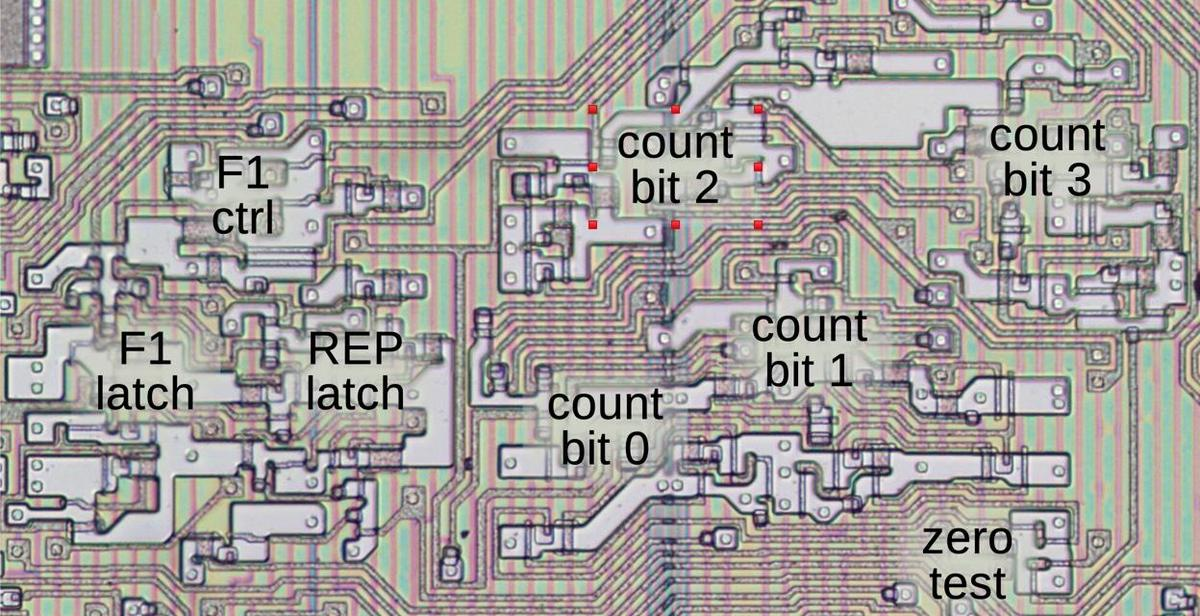The counter and F1 latch as they appear on the die. The latch for the REP state is also here.

The 8086 was pretty slow at multiplying compared to later Intel processors.13 The 8086 took up to 133 clock cycles to multiply unsigned 16-bit values due to the complicated microcode loops. By 1982, the Intel 286 processor cut this time down to 21 clock cycles. The Intel 486 (1989) used an improved algorithm that could end early, so multiplying by a small number could take just 9 cycles.

Although these optimizations improved performance, they still depended on looping over the bits. With the shift to 32-bit processors, the loop time became unwieldy. The solution was to replace the loop with hardware: instead of performing 32 shift-and-add loops, an array of adders could compute the multiplication in one step. This quantity of hardware was unreasonable in the 8086 era, but as Moore's law made transistors smaller and cheaper, hardware multiplication became practical. For instance, the Cyrix Cx486SLC (1992) had a 16-bit hardware multiplier that cut word multiply down to 3 cycles. The Intel Core 2 (2006) was even faster, able to complete a 32-bit multiplication every clock cycle.

Hardware multiplication is a fairly complicated subject, with many optimizations to maximize performance while minimizing hardware.14 Simply replacing the loop with a sequence of 32 adders is too slow because the result would be delayed while propagating through all the adders. The solution is to arrange the adders as a tree to provide parallelism. The first layer has 16 adders to add pairs of terms. The next layer adds pairs of these partial sums, and so forth. The resulting tree of adders is 5 layers deep rather than 32, reducing the time to compute the sum. Real multipliers achieve further performance improvements by splitting up the adders and creating a more complex tree: the venerable Wallace tree (1964) and Dadda multiplier (1965) are two popular approaches. Another optimization is the Booth algorithm (1951), which performs signed multiplication directly, without converting the arguments to positive values first. The Pentium 4 (2000) used a Booth encoder and a Wallace tree (ref), but research in the early 2000s found the Dadda tree is faster and it is now more popular.

## Conclusions

Multiplication is much harder to compute than addition or subtraction. The 8086 processor hid this complexity from the programmer by providing four multiplication instructions for byte and word multiplication of signed or unsigned values. These instructions implemented multiplication in microcode, performing shifts and adds in a loop. By using microcode subroutines and conditional execution, these four machine instructions share most of the microcode. As the microcode capacity of the 8086 was very small, this was a critical feature of the implementation.

If you made it through all the discussion of microcode, congratulations! Microcode is even harder to understand than assembly code. Part of the problem is that microcode is very fine-grain, with even ALU operations split into multiple steps. Another complication is that 8086 microcode performs a register move and another operation in parallel, so it's hard to keep track of what's going on. Microcode can seem a bit like a jigsaw puzzle, with pieces carefully fit together as compactly as possible. I hope the explanations here made sense, or at least gave you a feel for how microcode operates.

I've written multiple posts on the 8086 so far and plan to continue reverse-engineering the 8086 die so follow me on Twitter @kenshirriff or RSS for updates. I've also started experimenting with Mastodon recently as @[email protected].

## Notes and references

1. Mainframes going back to ENIAC had multiply and divide instructions. However, early microprocessors took a step back and didn't supports these more complex operations. (My theory is that the decline in memory prices made it more cost-effective to implement multiply and divide in software than hardware.) The National Semiconductor IMP-16, a 16-bit bit-slice microprocessor from 1973, may be the first with multiply and divide instructions. The 8-bit Motorola 6809 processor (1978) included 8-bit multiplication but not division. I think the 8086 was the first Intel processor to support multiplication.

2. The 8086 also supported division. Although the division instructions are similar to multiplication in many ways, I'm focusing on multiplication and ignoring division for this blog post.

3. My microcode analysis is based on Andrew Jenner's 8086 microcode disassembly

4. I think `CORX` stands for `Core Multiply` and `CORD` stands for `Core Divide`

5. The definitions of carry and overflow are different for multiplication compared to addition and subtraction. Note that the result of a multiplication operation will always fit in the available result space, which is twice as large as the arguments. For instance, the biggest value you can get by multiplying 16-bit values is `0xffff`×`0xffff`=`0xfffe0001` which fits into 32 bits. (Signed and 8-bit multiplications fit similarly.) This is in contrast to addition and subtraction, which can exceed their available space. A carry indicates that an addition exceeded its space when treated as unsigned, while an overflow indicates that an addition exceeded its space when treated as unsigned.

6. The Intel documentation states that the sign, carry, overflow, and parity flags are undefined after the `MUL` operation, even though the microcode causes them to be computed. The meaning of "undefined" is that programmers shouldn't count on the flag values because Intel might change the behavior in later chips. This thread discusses the effects of `MUL` on the flags, and how the behavior is different on the NEC V20 chip.

7. It may be worth explaining why the two's complement of a number is defined by adding 1 to the one's complement. The one's complement of a number simply flips all the bits. If you take a byte value `n`, `0xff` - `n` is the one's complement, since a 1 bit in `n` produces a 0 bit in the result.

Now, suppose we want to represent -5 as a signed byte. Adding `0x100` will keep the same byte value with a carry out of the byte. But `0x100` - 5 = (1 + 0xff) - 5 = 1 + (`0xff` - 5) = 1 + (one's complement of 5). Thus, it makes sense mathematically to represent -5 by adding 1 to the one's complement of 5, and this holds for any value.

8. The negation code is a bit tricky because the result is split across two words. In most cases, the upper word is bitwise complemented. However, if the lower word is zero, then the upper word is negated (two's complement). I'll demonstrate with 16-bit values to keep the examples small. The number 257 (0x0101) is negated to form -257 (0xfeff). Note that the upper byte is the one's complement (0x01 vs 0xfe) while the lower byte is two's complement (0x01 vs 0xff). On the other hand, the number 256 (0x0100) is negated to form -256 (0xff00). In this case, the upper byte is the two's complement (0x01 vs 0xff) and the lower byte is also the two's complement (0x00 vs 0x00).

(Mathematical explanation: the two's complement is formed by taking the one's complement and adding 1. In most cases, there won't be a carry from the low byte to the upper byte, so the upper byte will remain the one's complement. However, if the low byte is 0, the complement is 0xff and adding 1 will form a carry. Adding this carry to the upper byte yields the two's complement of that byte.)

To support multi-word negation, the 8086's `NEG` instruction clears the carry flag if the operand is 0, and otherwise sets the carry flag. (This is the opposite from the above because subtractions (including `NEG`) treat the carry flag as a borrow flag, with the opposite meaning.) The microcode `NEG` operation has identical behavior to the machine instruction, since it is used to implement the machine instruction.

Thus to perform a two-word negation, the microcode negates the low word (tmpC) and updates the flags (`F`). If the carry is set, the one's complement is applied to the upper word (tmpA). But if the carry is cleared, the two's complement is applied to tmpA.

9. The `IMULCOF` routine considers the upper half of the result significant if it is not the sign extension of the lower half. For instance, dropping the top byte of `0x0005` (+5) yields 0x05 (+5). Dropping the top byte of `0xfffb` (-5) yields 0xfb (-5). Thus, the upper byte is not significant in these cases. Conversely, dropping the top byte of `0x00fb` (+251) yields `0xfb` (-5), so the upper byte is significant.

10. Curiously, the 8086 patent states that the `X` register is a 4-bit register holding bits 3–6 of the byte (col. 9, line 20). But looking at the die, it is a 3-bit register holding bits 3–5 of the byte.

11. Some instructions are specified by bits 5–3 in the ModR/M byte rather than in the first opcode byte. The motivation is to avoid wasting bits for instructions that use a ModR/M byte but don't need a register specification. For instance, consider the instruction `ADD [BX],0x1234`. This instruction uses a ModR/M byte to specify the memory address. However, because it uses an immediate operand, it does not need the register specification normally provided by bits 5–3 of the ModR/M byte. This frees up the bits to specify the instruction. From one perspective, this is an ugly hack, while from another perspective it is a clever optimization.

12. Andrew Jenner discusses the F1 flag and the interaction between `REP` and multiplication here

13. Here are some detailed performance numbers. The 8086 processor takes 70–77 clock cycles to multiply 8-bit values and 118–133 clock cycles to multiply 16-bit values. Signed multiplies are a bit slower because of the sign calculations: 80–98 and 128–154 clock cycles respectively. The time is variable because of the conditional jumps in the multiplication process.

The Intel 186 (1982) optimized multiplication slightly, bringing the register word multiply down to 35–37 cycles. The Intel 286 (also 1982) reduced this to 21 clocks. The 486 (1989) used a shift-add multiply function but it had an "early out" algorithm that stopped when the remaining bits were zero, so a 16-bit multiply could take from 9 to 22 clocks. The 8087 floating point coprocessor (1980) used radix-4 multiplication, multiplying by pairs of bits at a time and either adding or subtracting. This yields half the addition cycles. The Pentium's P5 micro-architecture (1993) took the unusual approach of reusing the floating-point unit's hardware multiplier for integer multiplication, taking 10 cycles for a 32-bit multiplication.

14. This presentation gives a good overview of implementations of multiplication in hardware.